# RD Sharma Solutions for Class 8 Maths Chapter 25 Data Handling - III (Pictorial Representation of Data as Pie Charts or Circle Graphs)

In previous chapters, we have learnt about classification and tabulation of data and their graphical representation by using histograms. In this chapter, we shall learn about the diagramatic representation of data. There are a very large variety of diagrams in use, we mainly focus on pie-diagrams or pie-charts or circle graphs to represent the given data. By referring to RD Sharma Class 8 textbook students can get all the references required to solve the problems. Our experts have solved the problems using shortcut methods to help students understand the concepts clearly. RD Sharma Class 8 solutions are available in the pdf format, which can be downloaded easily from the links provided below.

Chapter 25 Data Handling – III (Pictorial Representation of Data as Pie Charts or Circle Graphs) contains two exercises and the RD Sharma Class 8 Solutions present in this page provide solutions to the questions present in each exercise. Now, let us have a look at the concepts discussed in this chapter.

• Pie-diagrams.
• Construction of Pie-diagrams.
• Reading of Pie-charts.

## Download the Pdf of RD Sharma Solutions for Class 8 Maths Chapter 25 Data Handling – III (Pictorial Representation of Data as Pie Charts or Circle Graphs)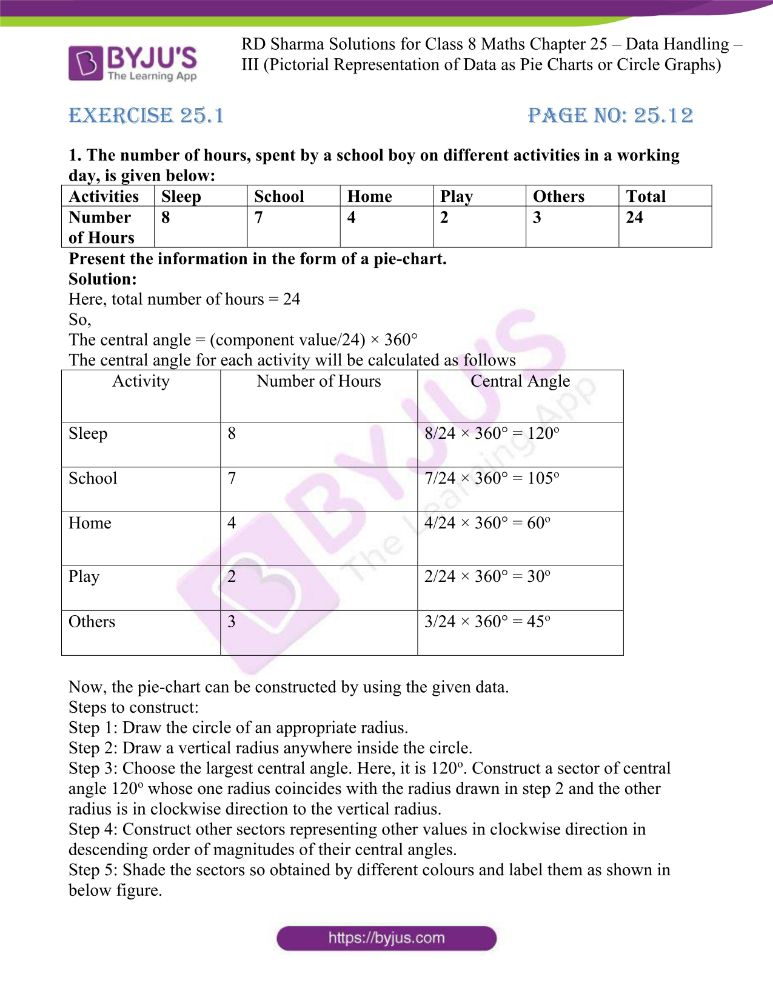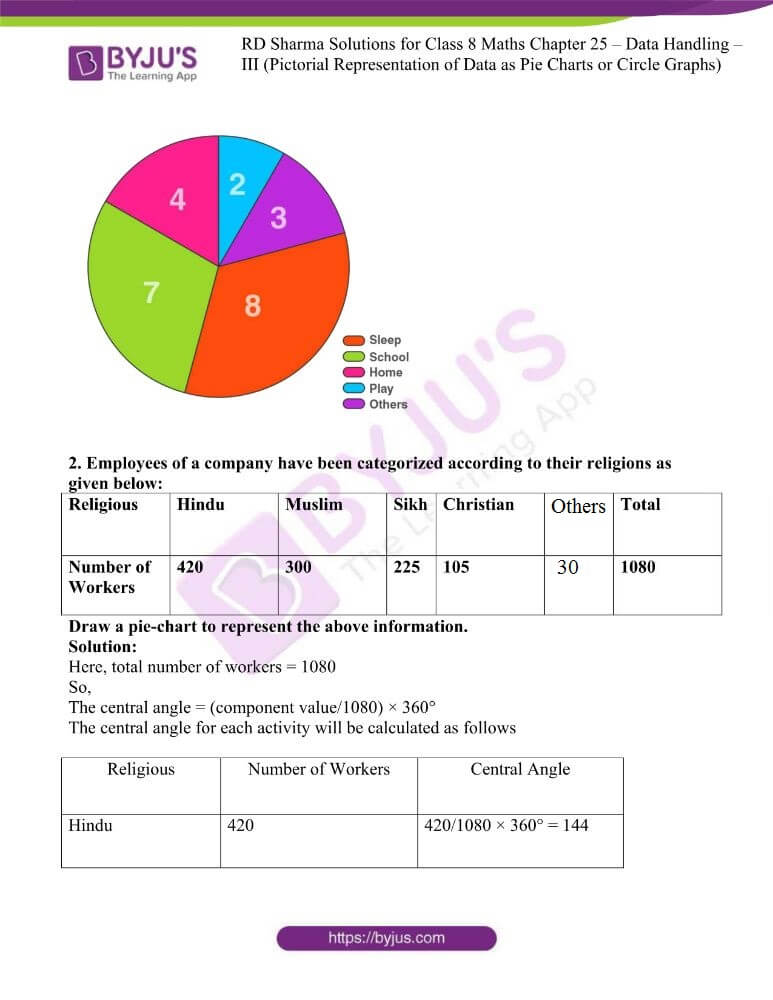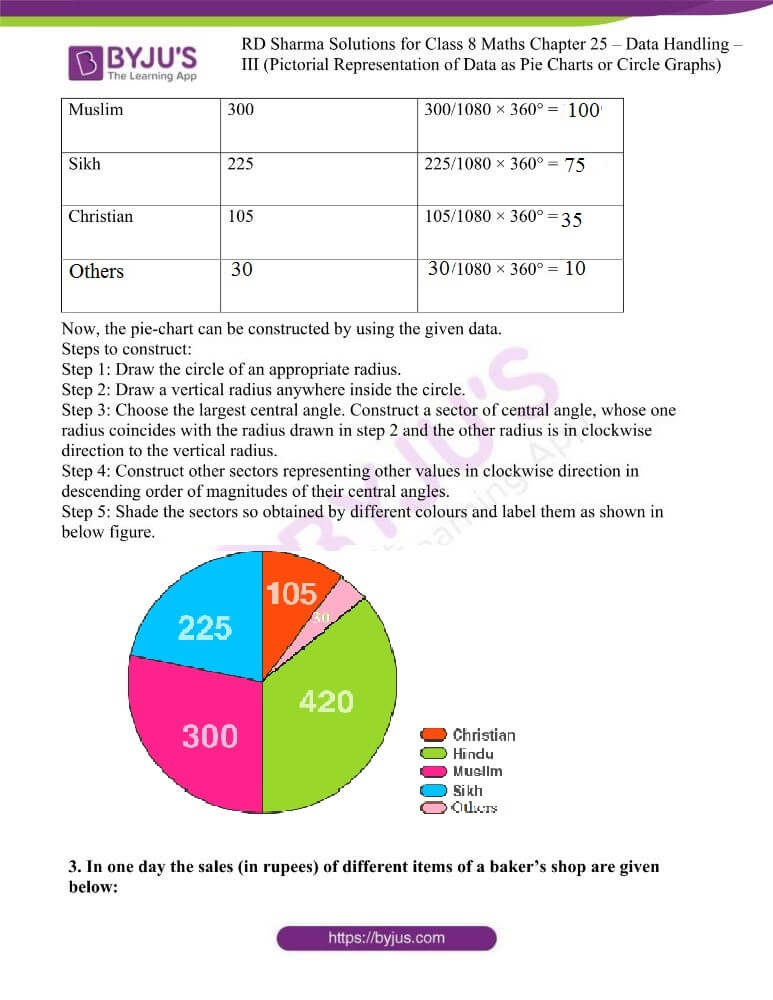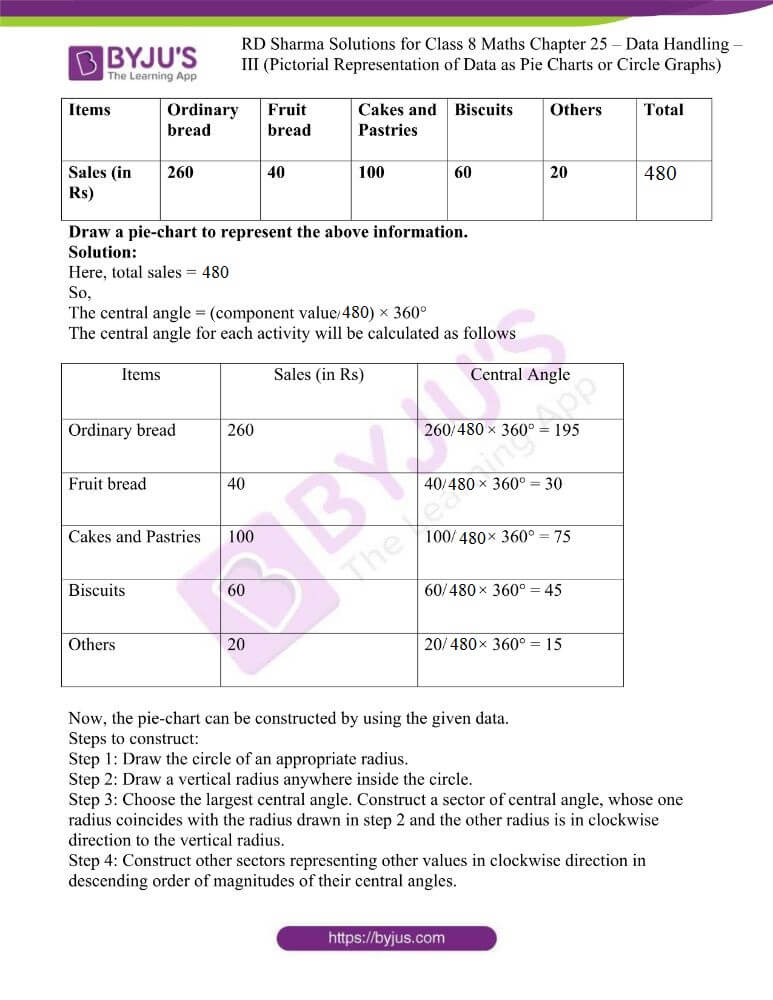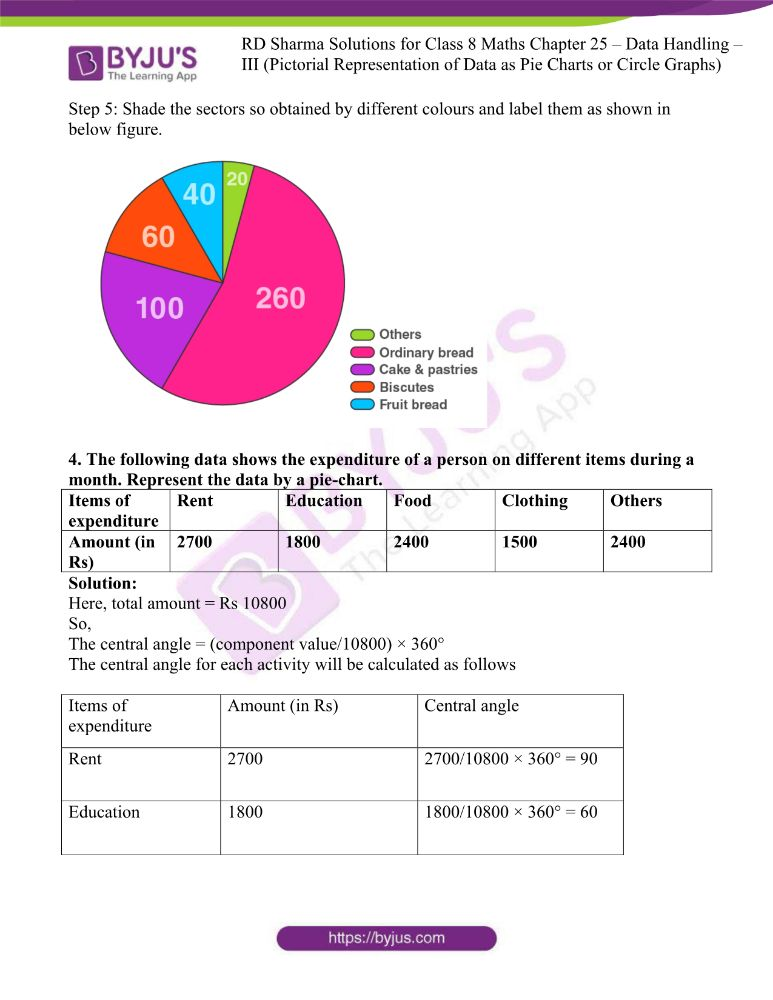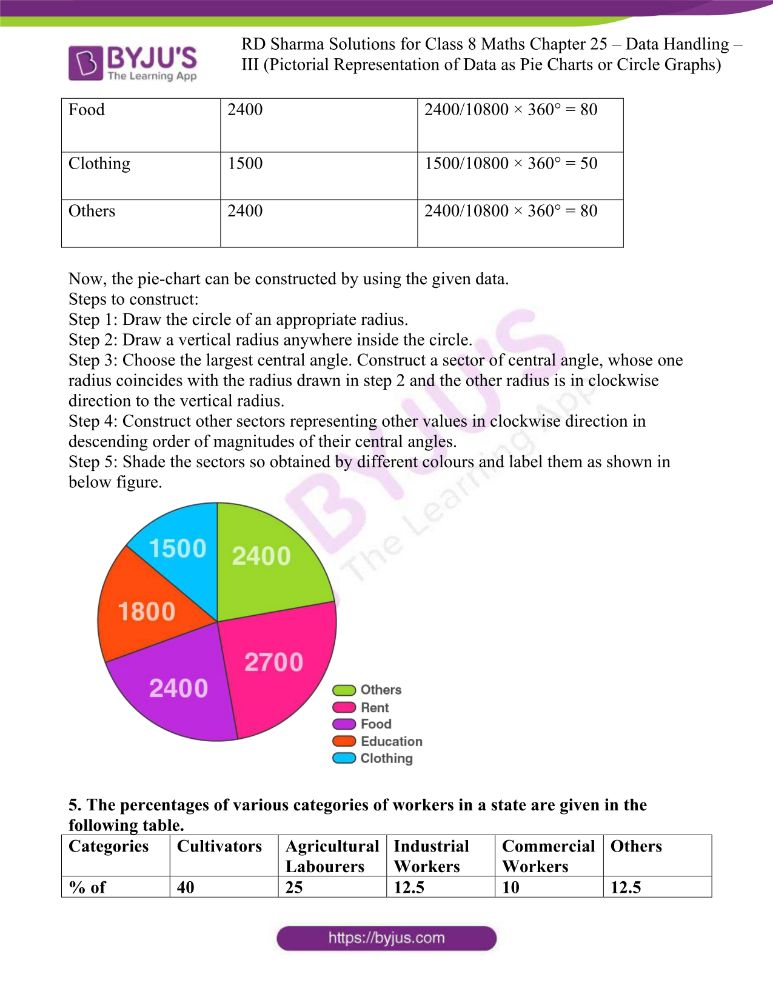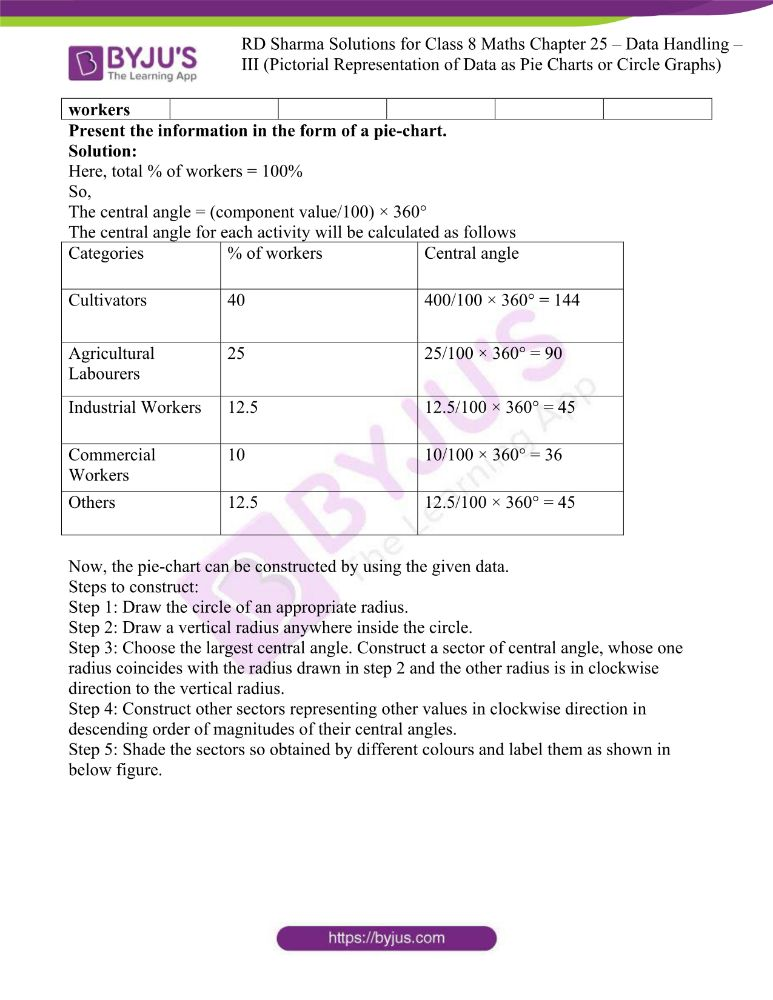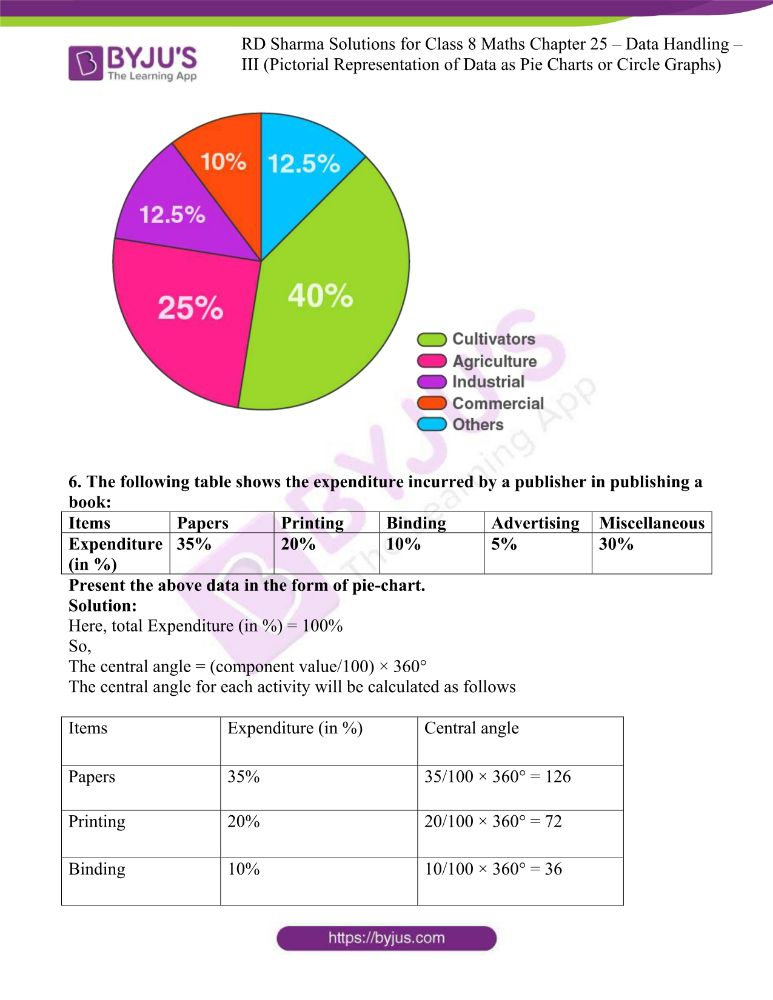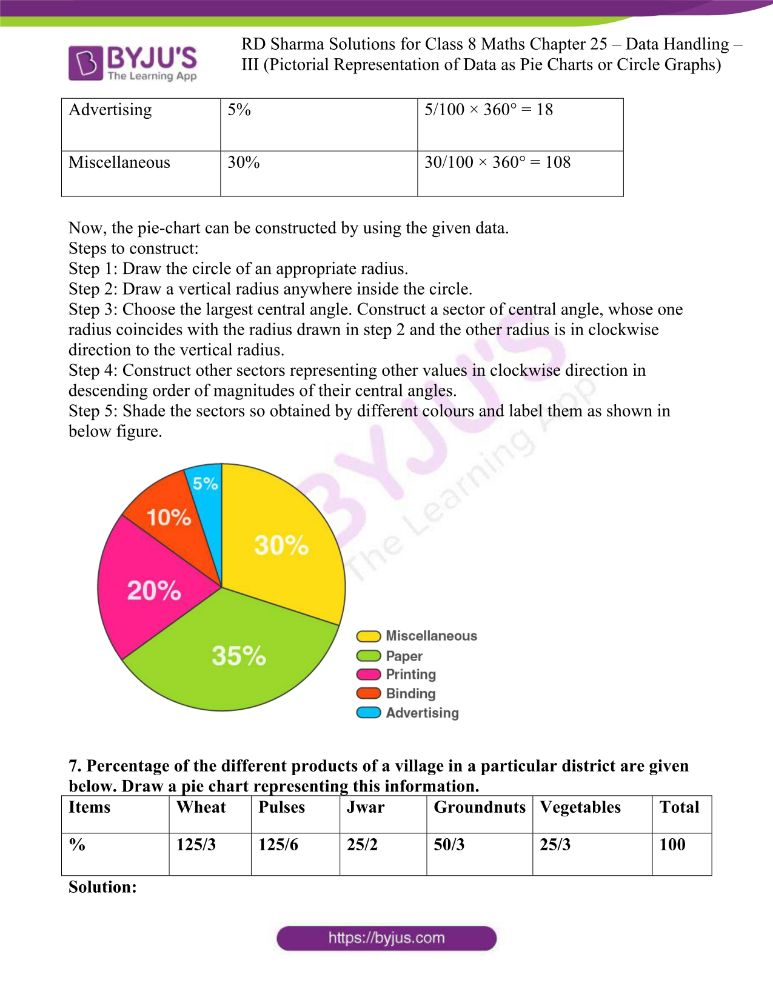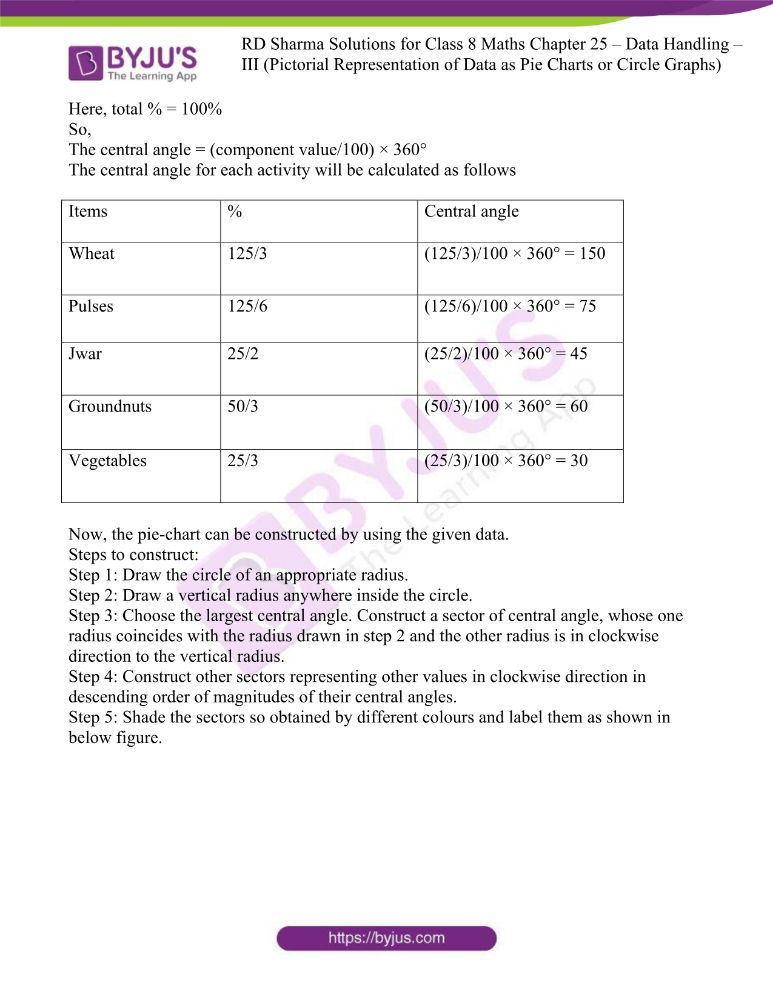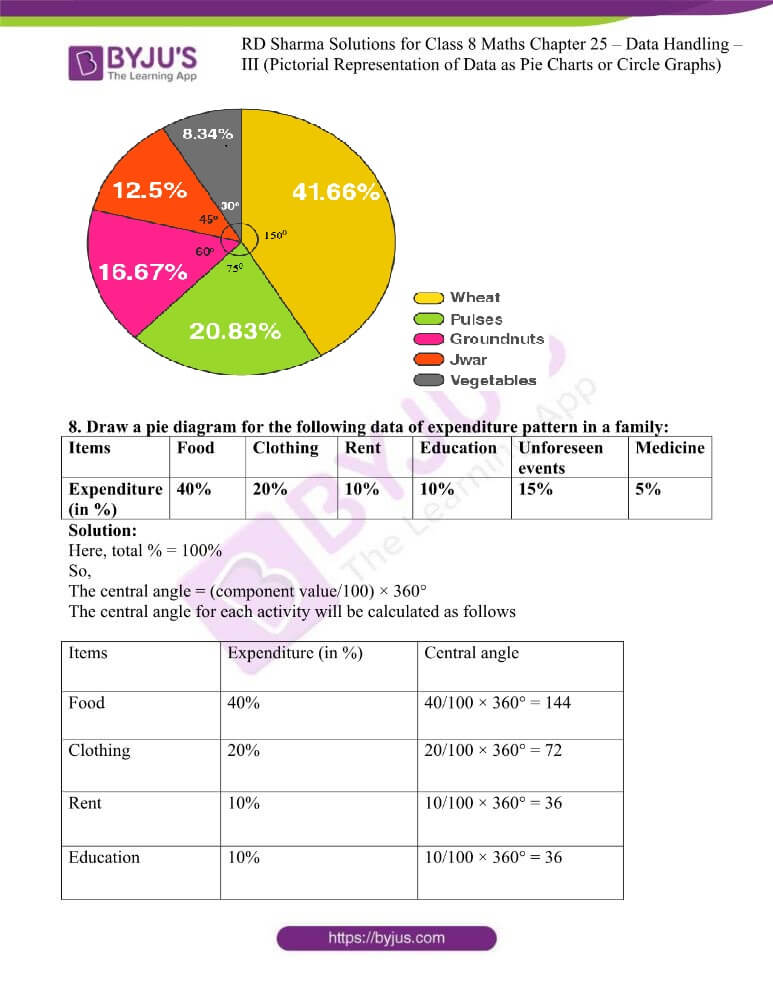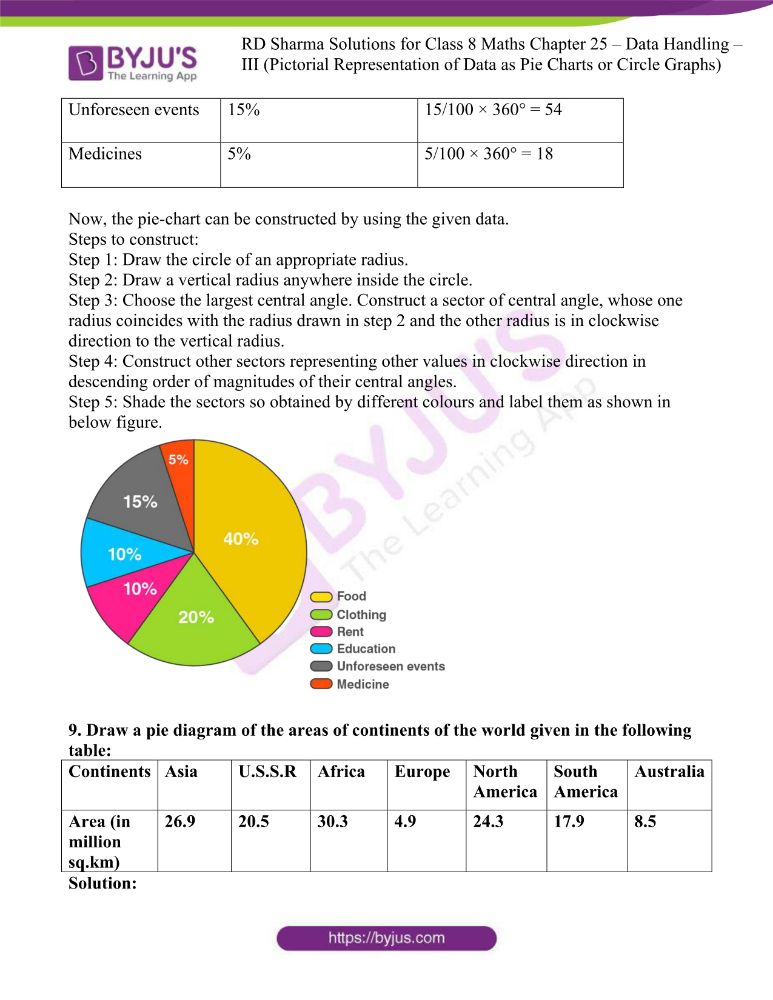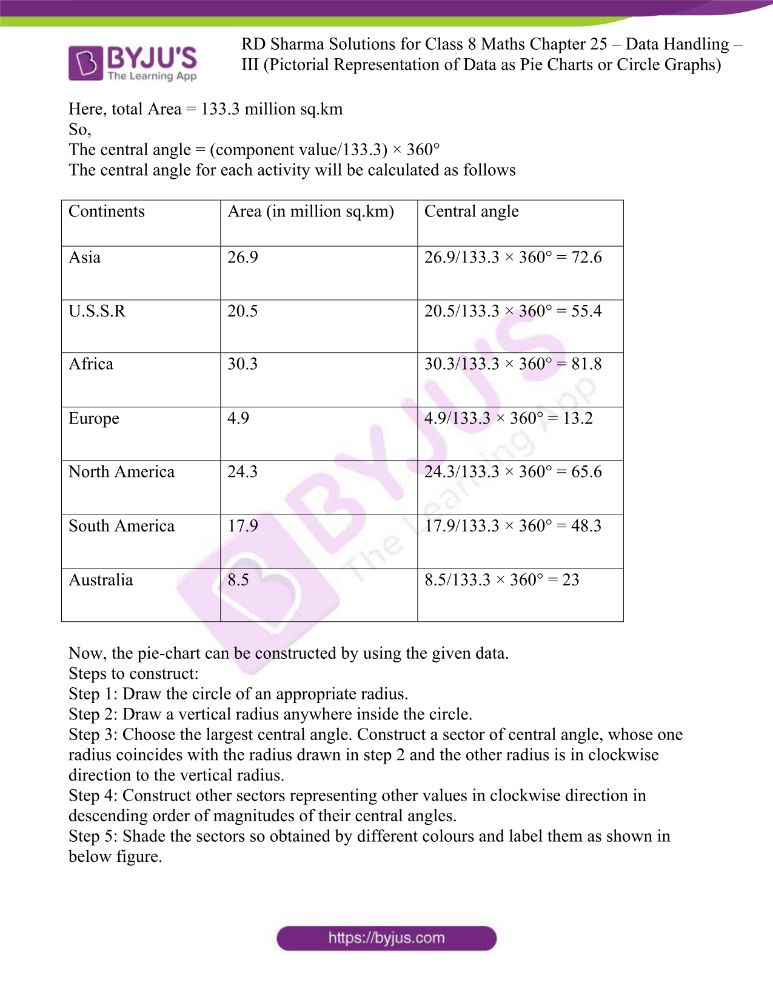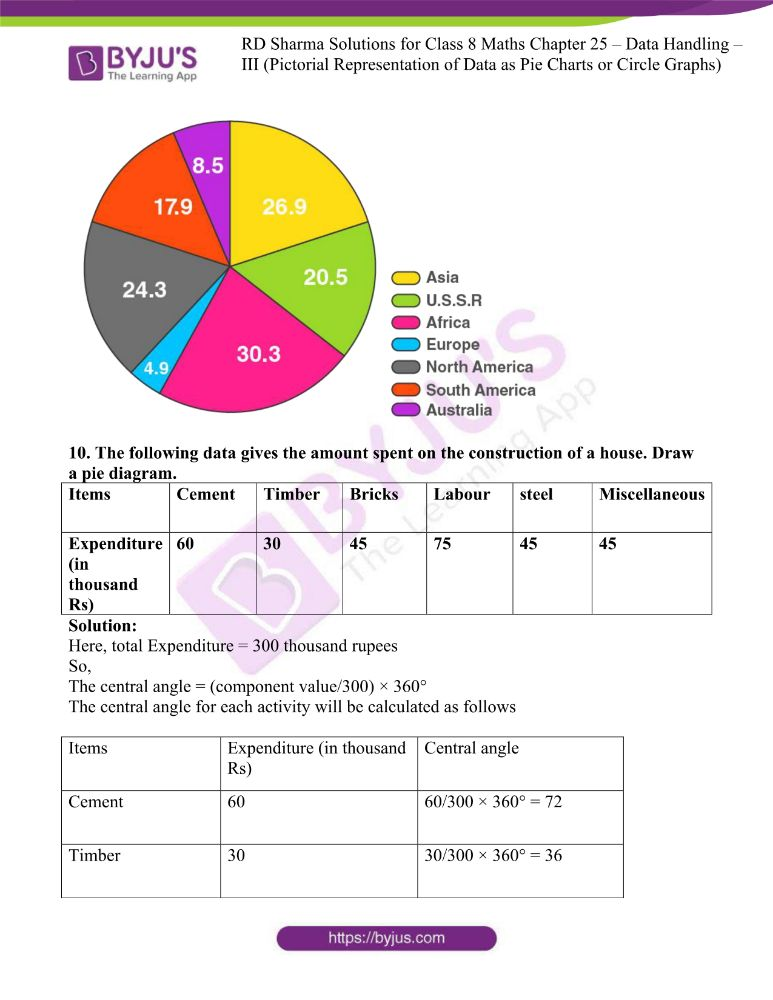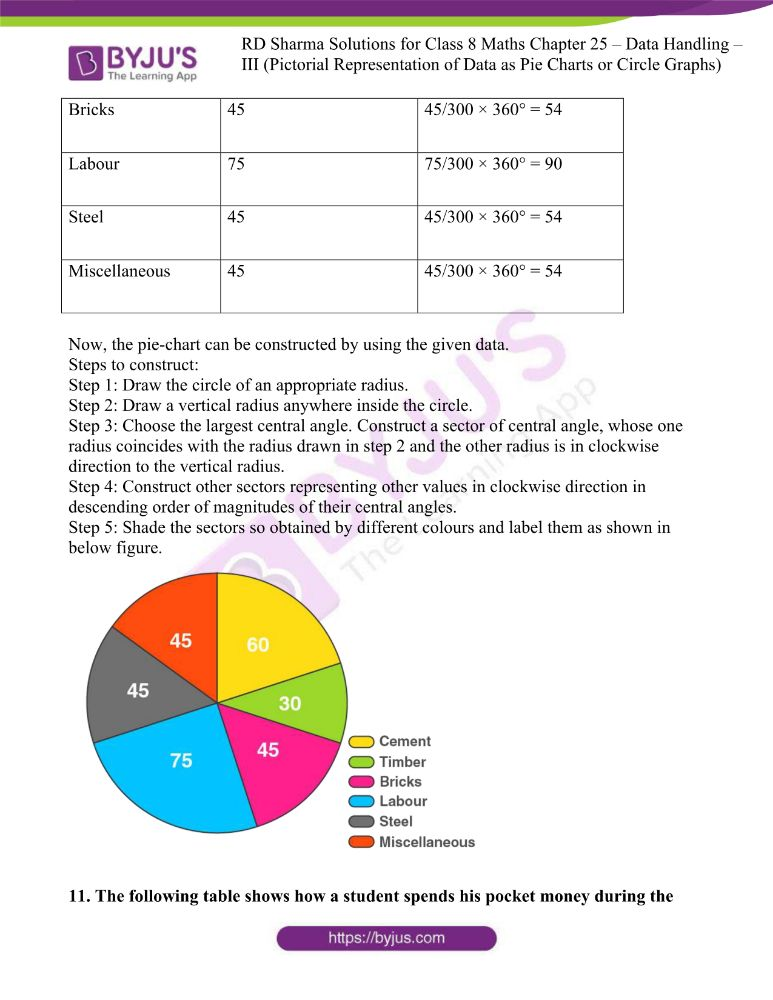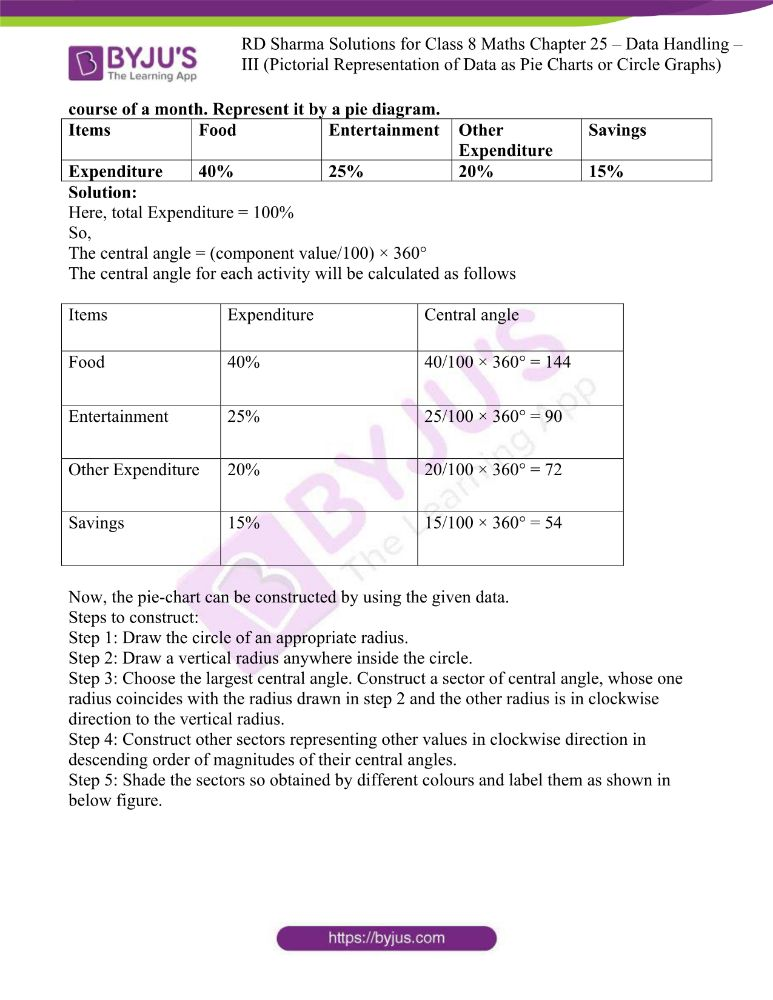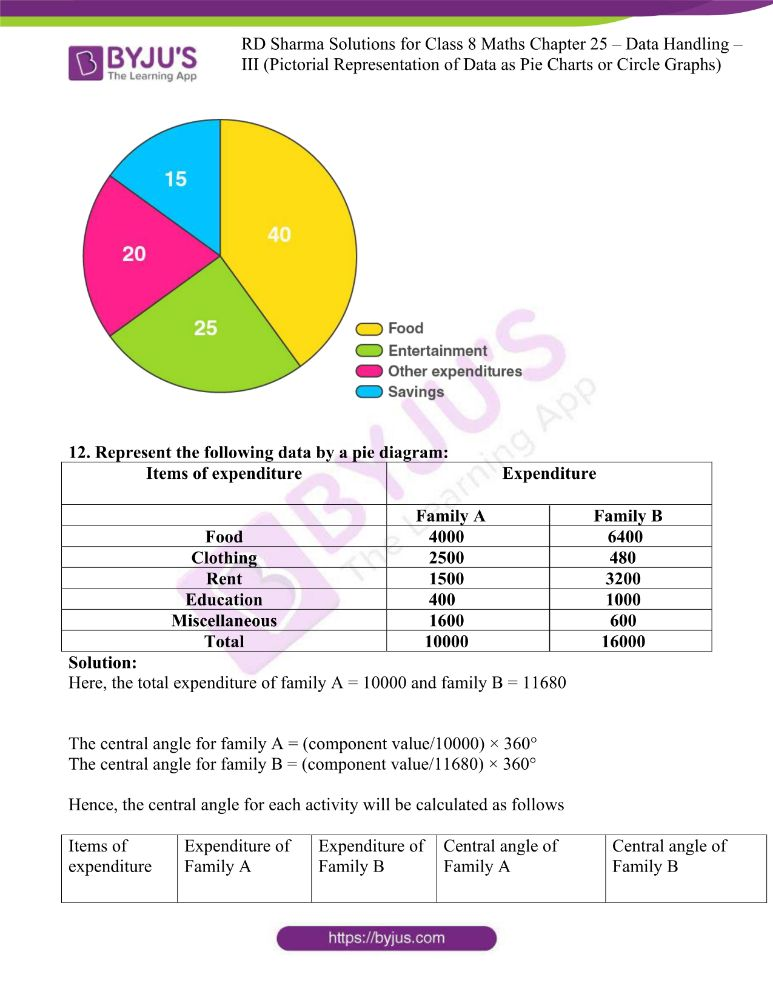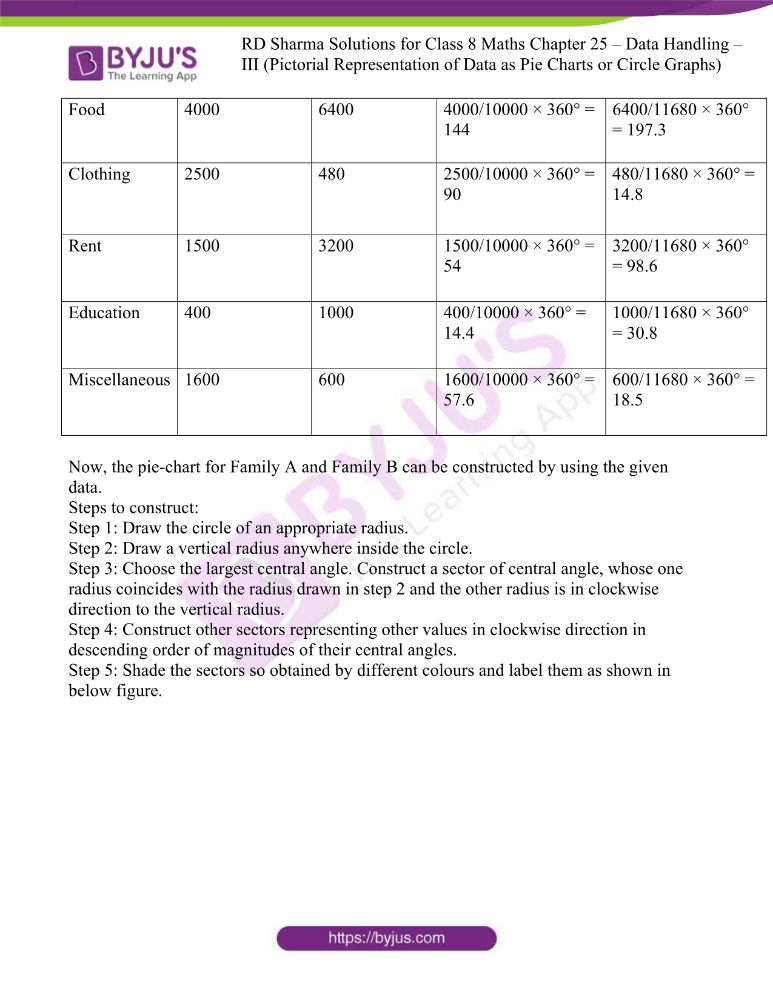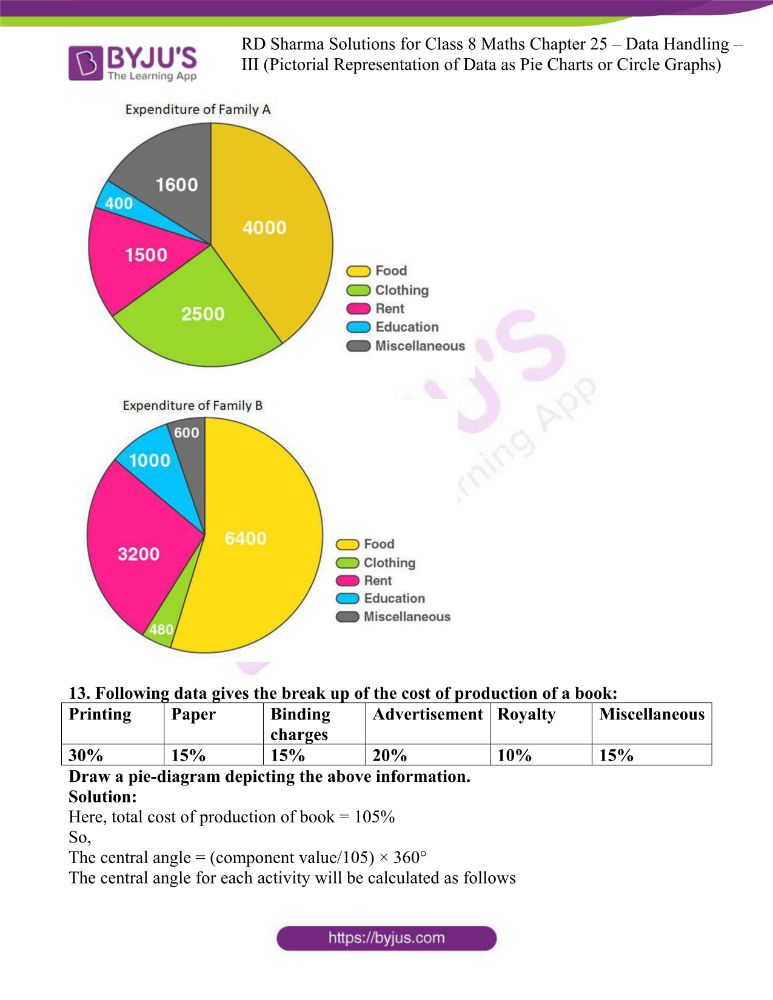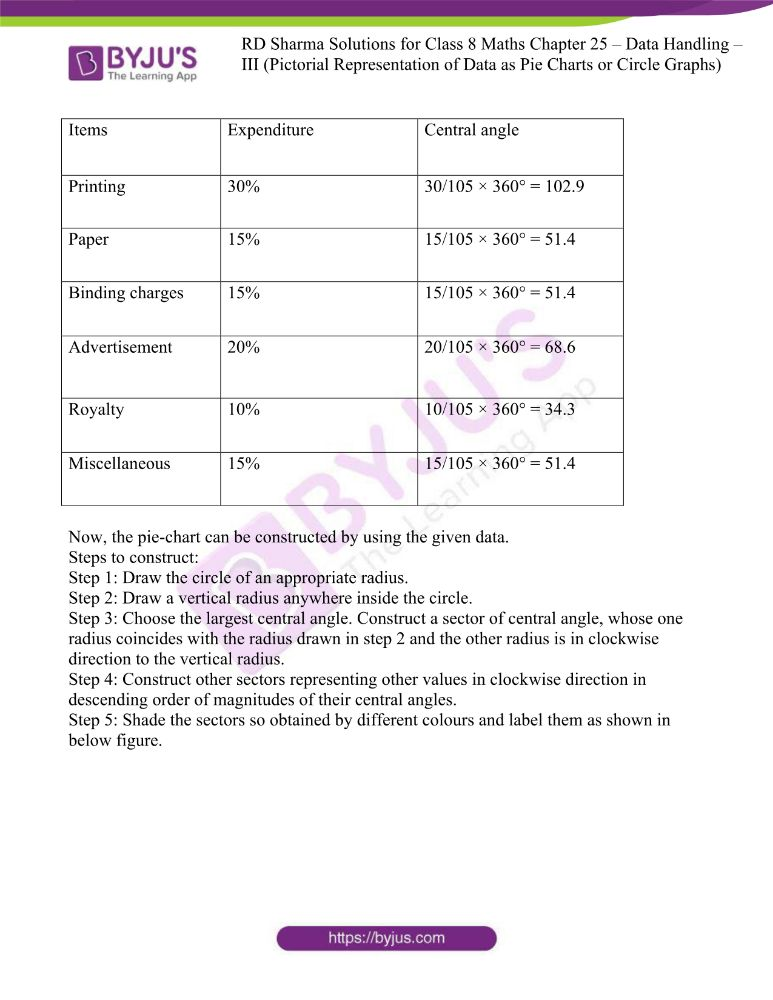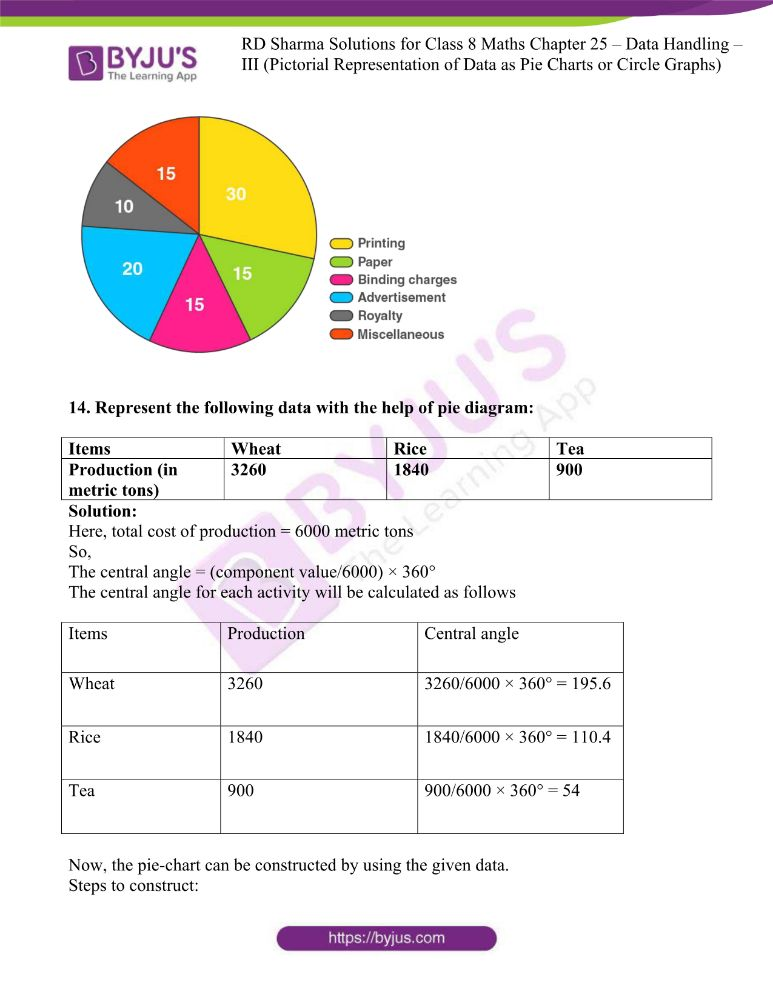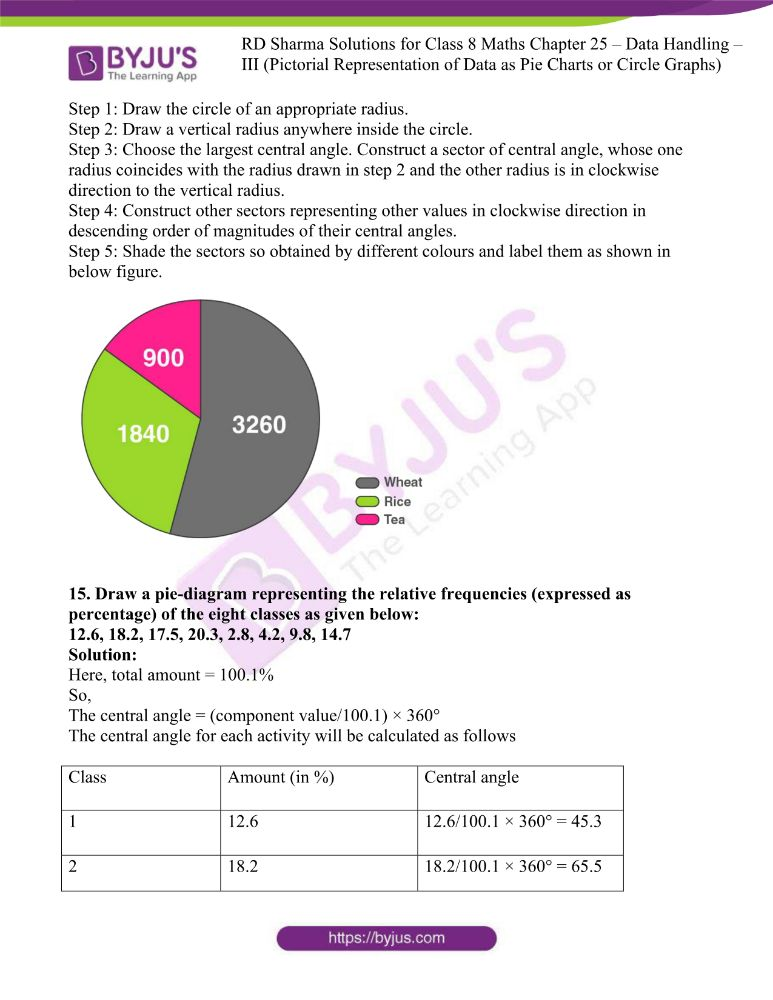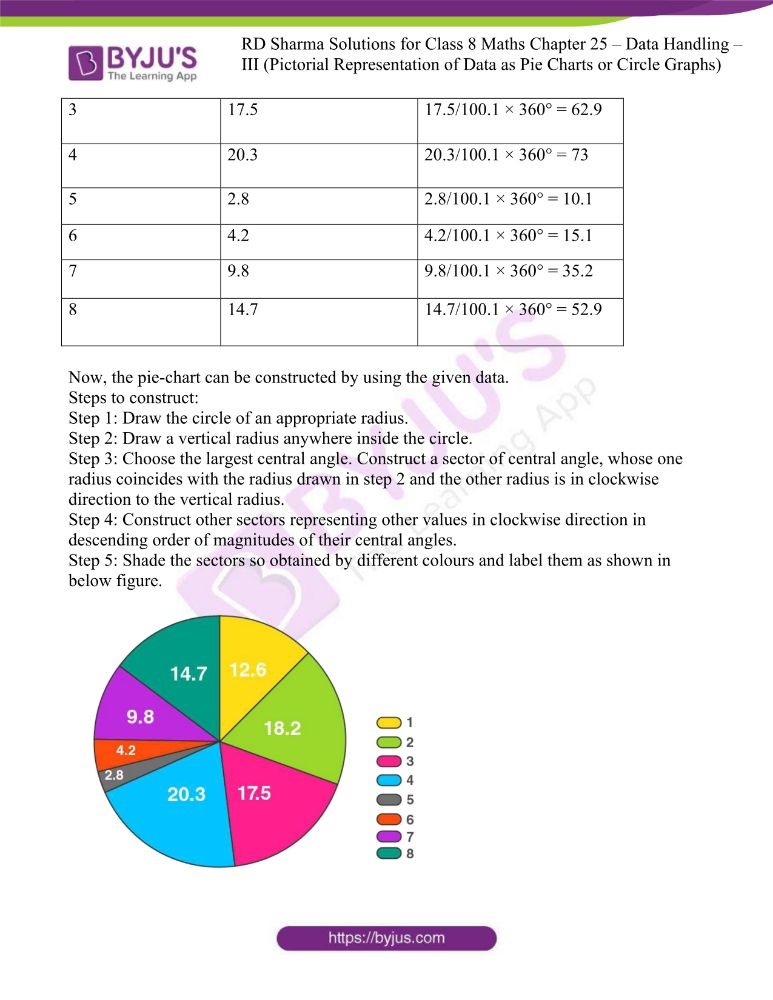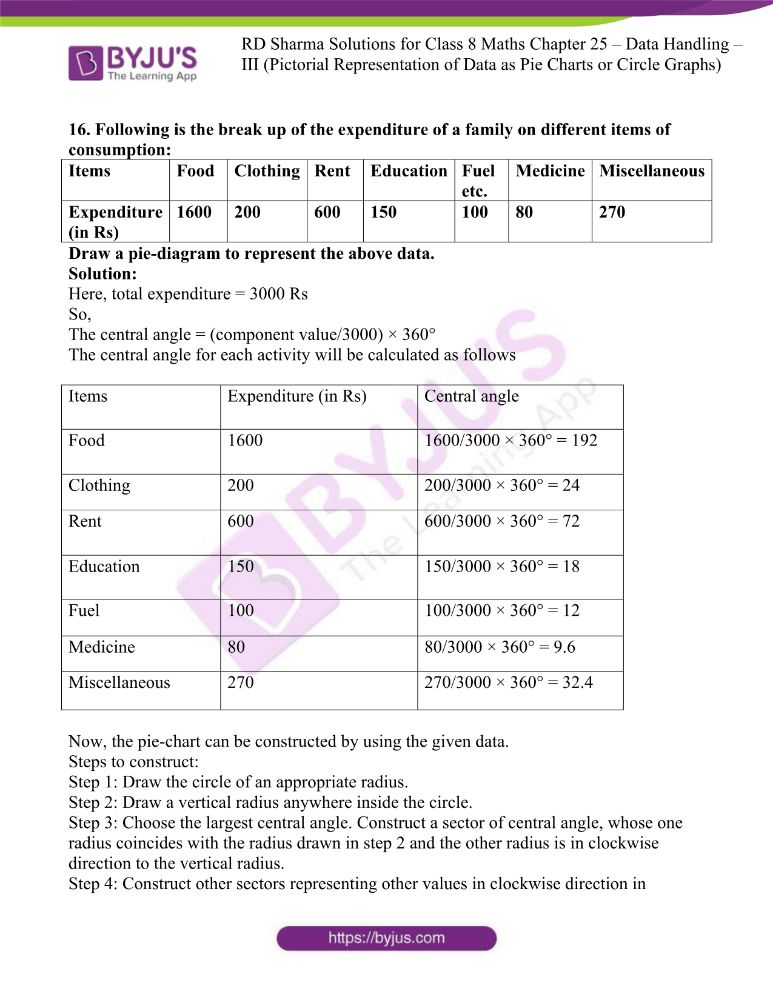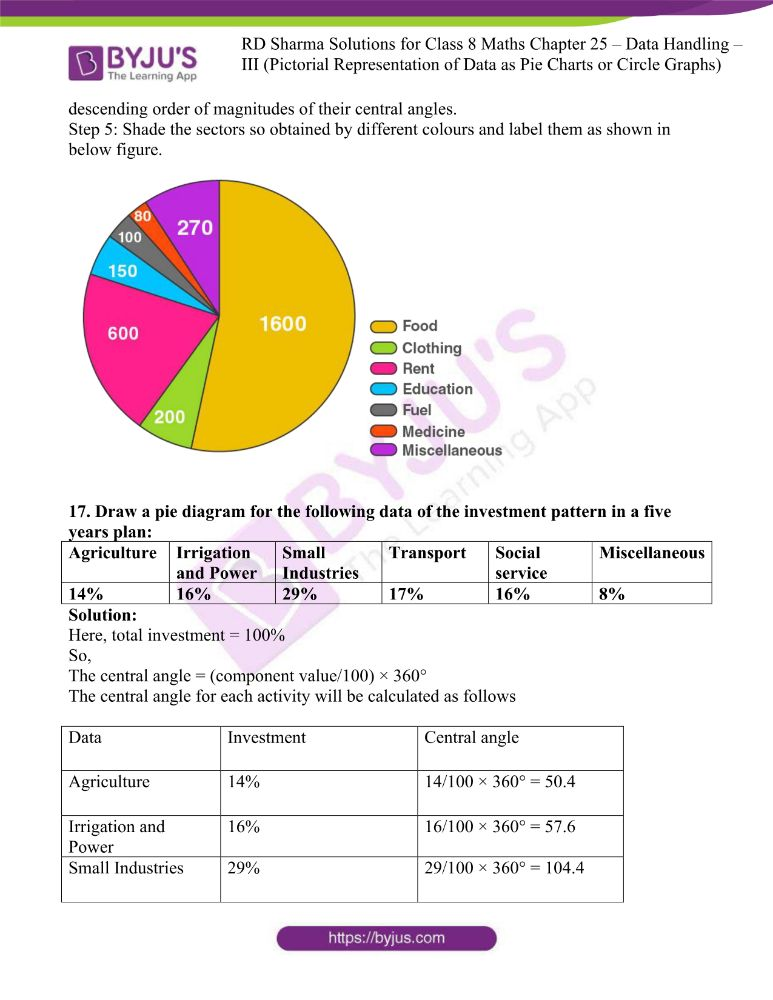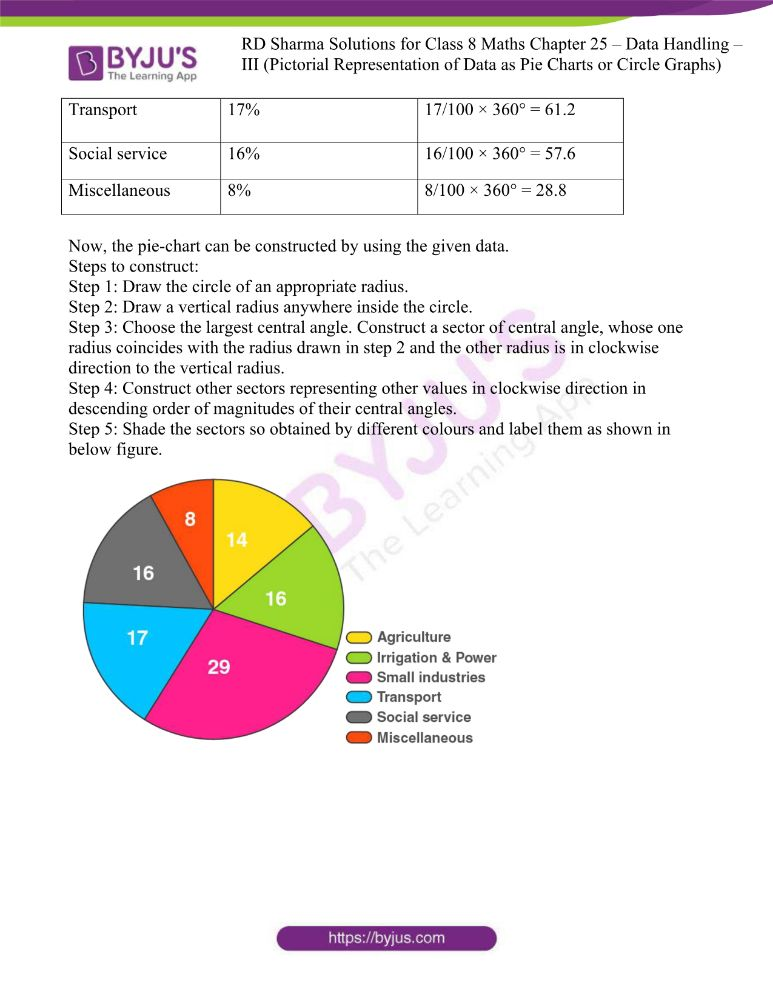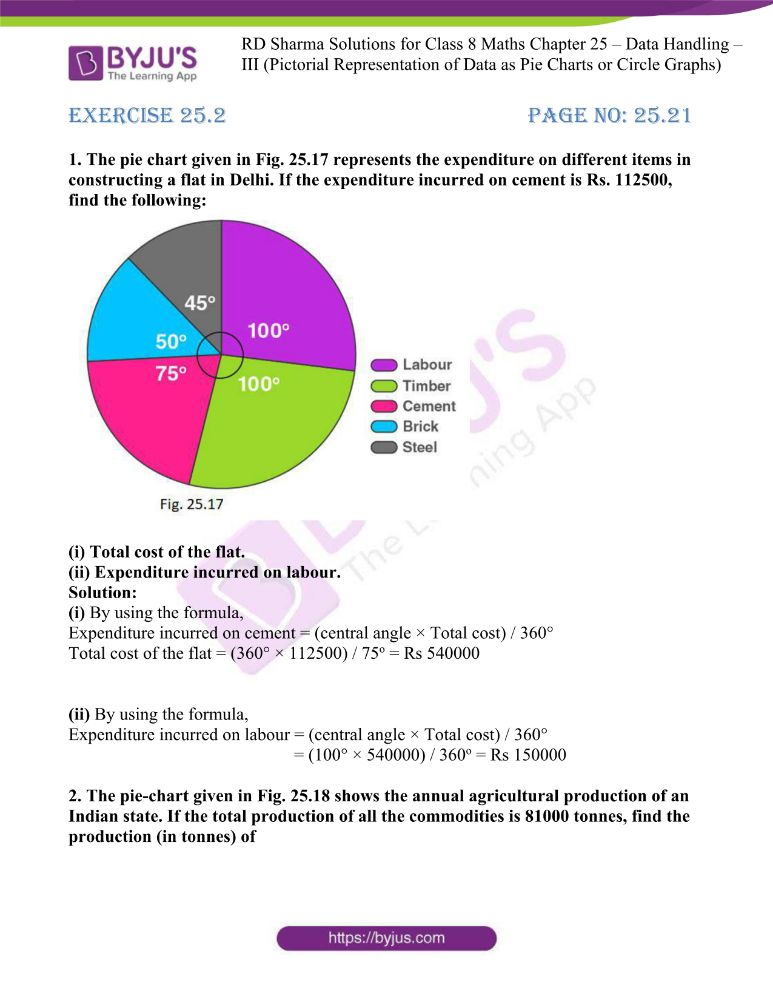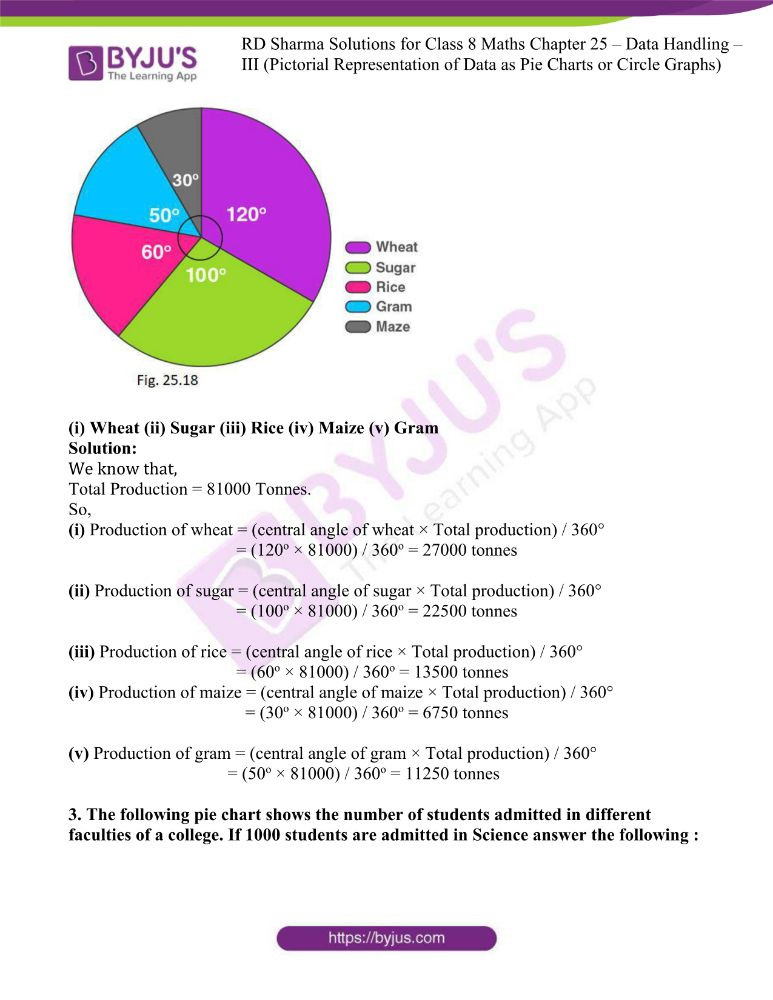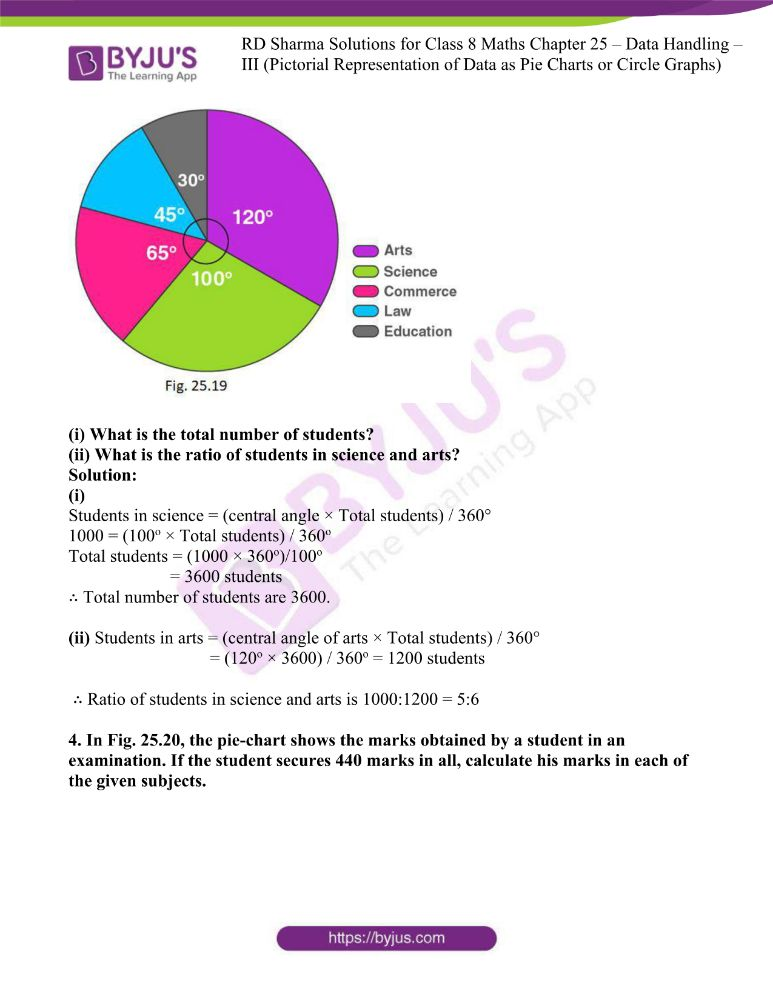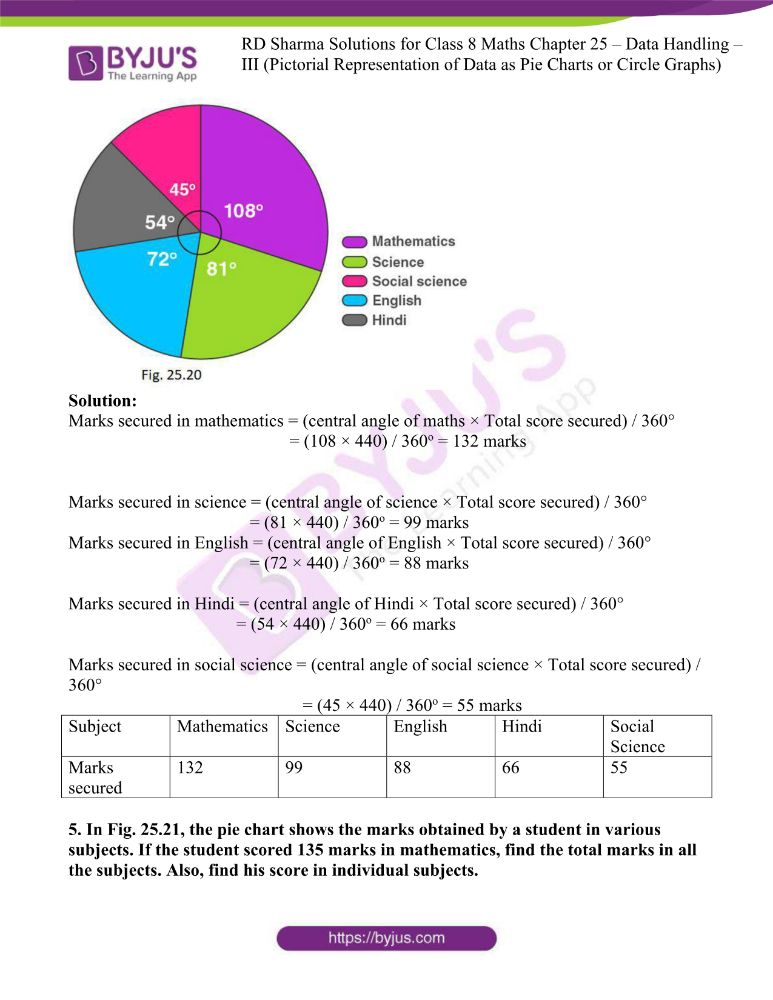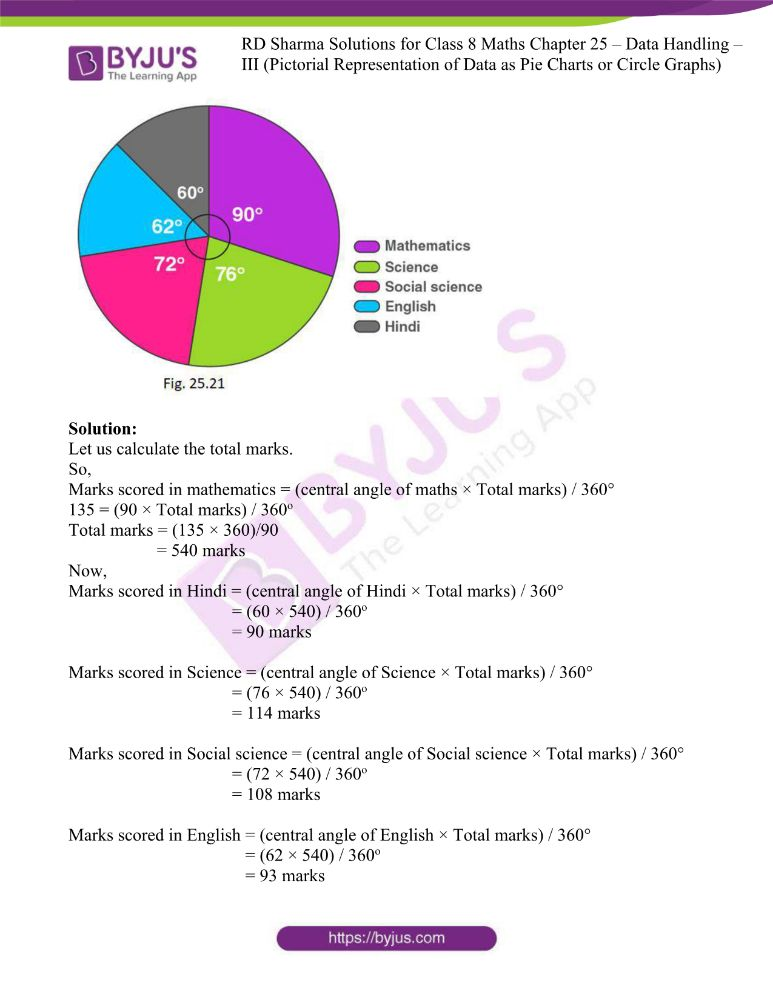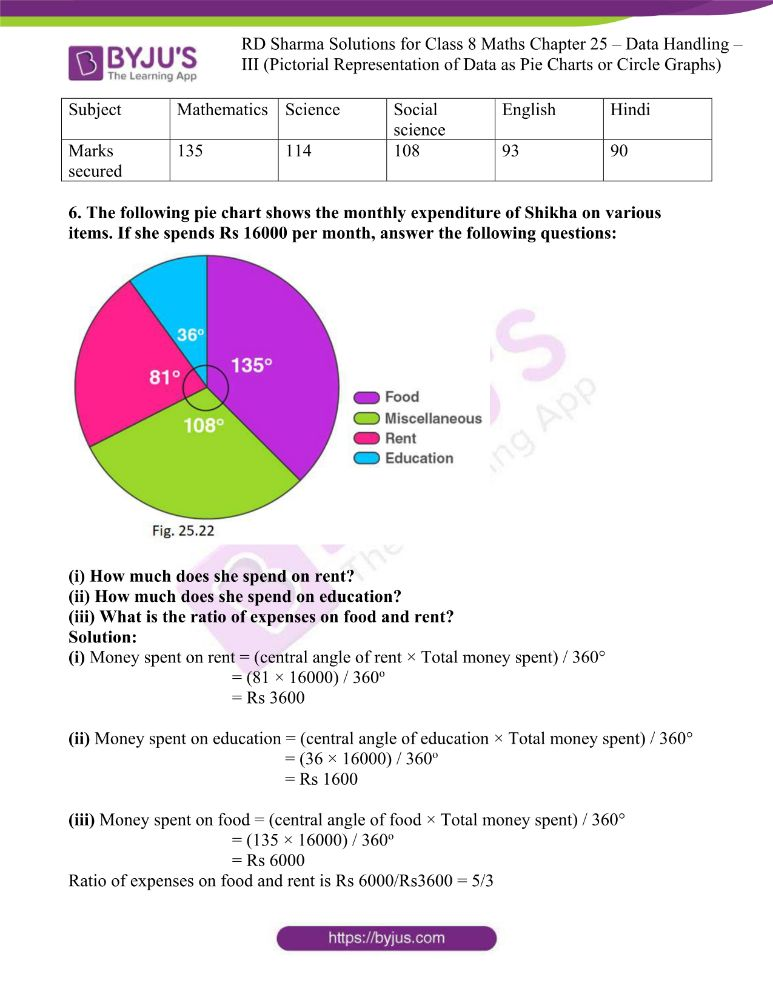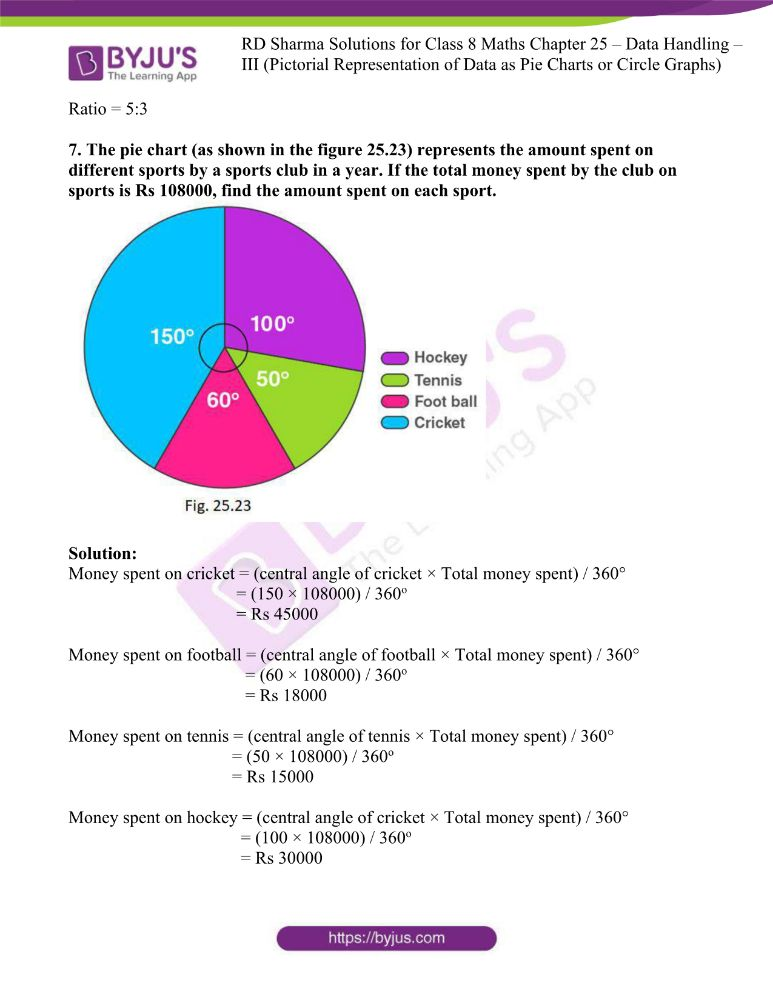### Access answers to Maths RD Sharma Solutions For Class 8 Chapter 25 Data Handling – III (Pictorial Representation of Data as Pie Charts or Circle Graphs)

EXERCISE 25.1 PAGE NO: 25.12

1. The number of hours, spent by a school boy on different activities in a working day, is given below:

 Activities Sleep School Home Play Others Total Number of Hours 8 7 4 2 3 24

Present the information in the form of a pie-chart.

Solution:

Here, total number of hours = 24

So,

The central angle = (component value/24) × 360°

The central angle for each activity will be calculated as follows

 Activity Number of Hours Central Angle Sleep 8 8/24 × 360° = 120o School 7 7/24 × 360° = 105o Home 4 4/24 × 360° = 60o Play 2 2/24 × 360° = 30o Others 3 3/24 × 360° = 45o

Now, the pie-chart can be constructed by using the given data.

Steps to construct:

Step 1: Draw the circle of an appropriate radius.

Step 2: Draw a vertical radius anywhere inside the circle.

Step 3: Choose the largest central angle. Here, it is 120o. Construct a sector of central angle 120o whose one radius coincides with the radius drawn in step 2 and the other radius is in clockwise direction to the vertical radius.

Step 4: Construct other sectors representing other values in clockwise direction in descending order of magnitudes of their central angles.

Step 5: Shade the sectors so obtained by different colours and label them as shown in below figure.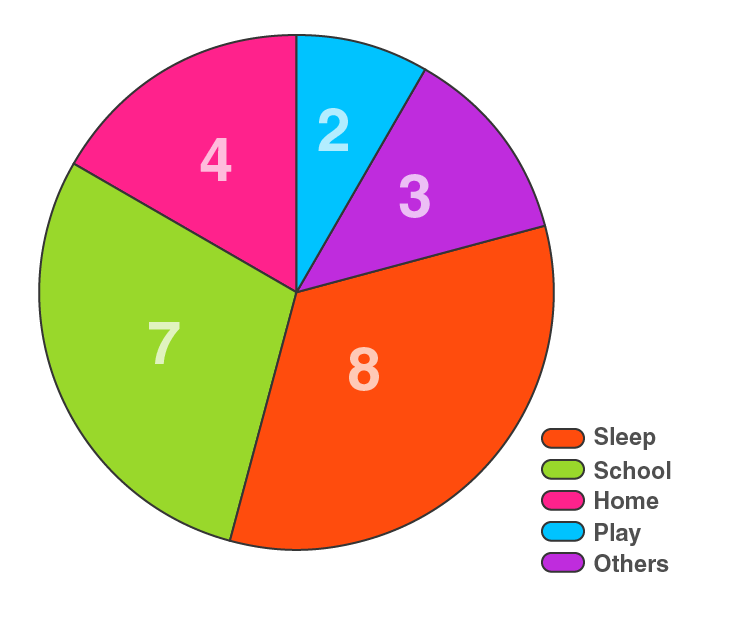2. Employees of a company have been categorized according to their religions as given below:

 Religious Hindu Muslim Sikh Christian Others Total Number of Workers 420 300 225 105 30 1080

Draw a pie-chart to represent the above information.

Solution:

Here, total number of workers = 1080

So,

The central angle = (component value/1080) × 360°

The central angle for each activity will be calculated as follows

 Religious Number of Workers Central Angle Hindu 420 420/1080 × 360° = 144 Muslim 300 300/1080 × 360° = 102.9 Sikh 225 225/1080 × 360° = 77.14 Christian 105 105/1080 × 360° = 36 Others 30 30/1080 × 360° = 10

Now, the pie-chart can be constructed by using the given data.

Steps to construct:

Step 1: Draw the circle of an appropriate radius.

Step 2: Draw a vertical radius anywhere inside the circle.

Step 3: Choose the largest central angle. Construct a sector of central angle, whose one radius coincides with the radius drawn in step 2 and the other radius is in clockwise direction to the vertical radius.

Step 4: Construct other sectors representing other values in clockwise direction in descending order of magnitudes of their central angles.

Step 5: Shade the sectors so obtained by different colours and label them as shown in below figure.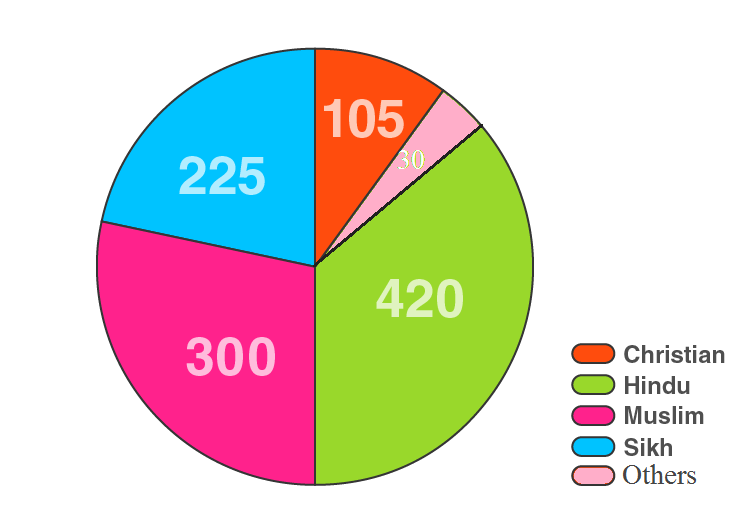3. In one day the sales (in rupees) of different items of a baker’s shop are given below:

 Items Ordinary bread Fruit bread Cakes and Pastries Biscuits Others Total Sales (in Rs) 260 40 100 60 20 480

Draw a pie-chart to represent the above information.

Solution:

Here, total sales = 480

So,

The central angle = (component value/480) × 360°

The central angle for each activity will be calculated as follows

 Items Sales (in Rs) Central Angle Ordinary bread 260 260/480 × 360° = 195 Fruit bread 40 40/480 × 360° = 30 Cakes and Pastries 100 100/480 × 360° = 75 Biscuits 60 60/480 × 360° = 45 Others 20 20/480 × 360° = 15

Now, the pie-chart can be constructed by using the given data.

Steps to construct:

Step 1: Draw the circle of an appropriate radius.

Step 2: Draw a vertical radius anywhere inside the circle.

Step 3: Choose the largest central angle. Construct a sector of central angle, whose one radius coincides with the radius drawn in step 2 and the other radius is in clockwise direction to the vertical radius.

Step 4: Construct other sectors representing other values in clockwise direction in descending order of magnitudes of their central angles.

Step 5: Shade the sectors so obtained by different colours and label them as shown in below figure.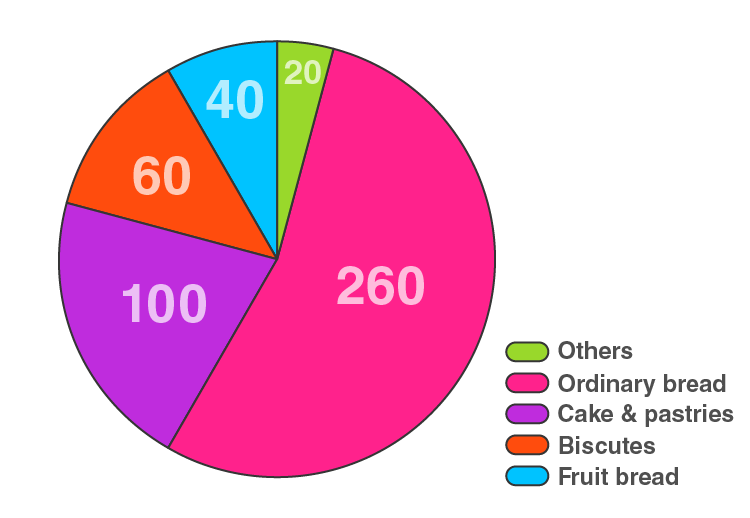4. The following data shows the expenditure of a person on different items during a month. Represent the data by a pie-chart.

 Items of expenditure Rent Education Food Clothing Others Amount (in Rs) 2700 1800 2400 1500 2400

Solution:

Here, total amount = Rs 10800

So,

The central angle = (component value/10800) × 360°

The central angle for each activity will be calculated as follows

 Items of expenditure Amount (in Rs) Central angle Rent 2700 2700/10800 × 360° = 90 Education 1800 1800/10800 × 360° = 60 Food 2400 2400/10800 × 360° = 80 Clothing 1500 1500/10800 × 360° = 50 Others 2400 2400/10800 × 360° = 80

Now, the pie-chart can be constructed by using the given data.

Steps to construct:

Step 1: Draw the circle of an appropriate radius.

Step 2: Draw a vertical radius anywhere inside the circle.

Step 3: Choose the largest central angle. Construct a sector of central angle, whose one radius coincides with the radius drawn in step 2 and the other radius is in clockwise direction to the vertical radius.

Step 4: Construct other sectors representing other values in clockwise direction in descending order of magnitudes of their central angles.

Step 5: Shade the sectors so obtained by different colours and label them as shown in below figure.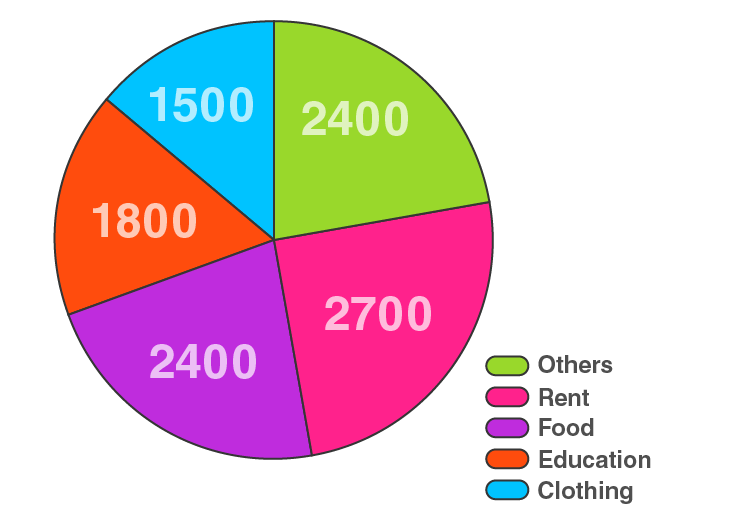5. The percentages of various categories of workers in a state are given in the following table.

 Categories Cultivators Agricultural Labourers Industrial Workers Commercial Workers Others % of workers 40 25 12.5 10 12.5

Present the information in the form of a pie-chart.

Solution:

Here, total % of workers = 100%

So,

The central angle = (component value/100) × 360°

The central angle for each activity will be calculated as follows

 Categories % of workers Central angle Cultivators 40 400/100 × 360° = 144 Agricultural Labourers 25 25/100 × 360° = 90 Industrial Workers 12.5 12.5/100 × 360° = 45 Commercial Workers 10 10/100 × 360° = 36 Others 12.5 12.5/100 × 360° = 45

Now, the pie-chart can be constructed by using the given data.

Steps to construct:

Step 1: Draw the circle of an appropriate radius.

Step 2: Draw a vertical radius anywhere inside the circle.

Step 3: Choose the largest central angle. Construct a sector of central angle, whose one radius coincides with the radius drawn in step 2 and the other radius is in clockwise direction to the vertical radius.

Step 4: Construct other sectors representing other values in clockwise direction in descending order of magnitudes of their central angles.

Step 5: Shade the sectors so obtained by different colours and label them as shown in below figure.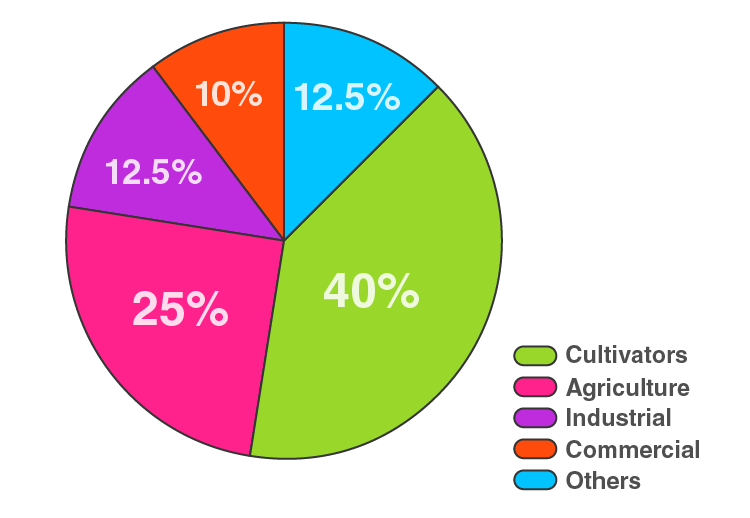6. The following table shows the expenditure incurred by a publisher in publishing a book:

 Items Papers Printing Binding Advertising Miscellaneous Expenditure (in %) 35% 20% 10% 5% 30%

Present the above data in the form of pie-chart.

Solution:

Here, total Expenditure (in %) = 100%

So,

The central angle = (component value/100) × 360°

The central angle for each activity will be calculated as follows

 Items Expenditure (in %) Central angle Papers 35% 35/100 × 360° = 126 Printing 20% 20/100 × 360° = 72 Binding 10% 10/100 × 360° = 36 Advertising 5% 5/100 × 360° = 18 Miscellaneous 30% 30/100 × 360° = 108

Now, the pie-chart can be constructed by using the given data.

Steps to construct:

Step 1: Draw the circle of an appropriate radius.

Step 2: Draw a vertical radius anywhere inside the circle.

Step 3: Choose the largest central angle. Construct a sector of central angle, whose one radius coincides with the radius drawn in step 2 and the other radius is in clockwise direction to the vertical radius.

Step 4: Construct other sectors representing other values in clockwise direction in descending order of magnitudes of their central angles.

Step 5: Shade the sectors so obtained by different colours and label them as shown in below figure.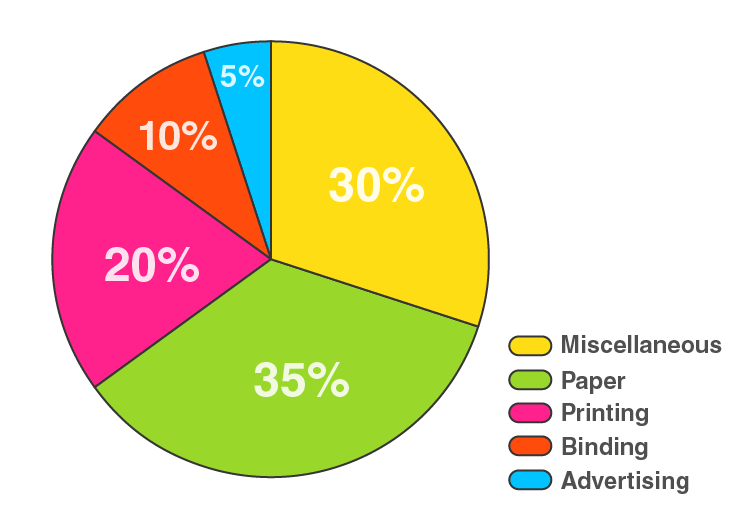7. Percentage of the different products of a village in a particular district are given below. Draw a pie chart representing this information.

 Items Wheat Pulses Jwar Groundnuts Vegetables Total % 125/3 125/6 25/2 50/3 25/3 100

Solution:

Here, total % = 100%

So,

The central angle = (component value/100) × 360°

The central angle for each activity will be calculated as follows

 Items % Central angle Wheat 125/3 (125/3)/100 × 360° = 150 Pulses 125/6 (125/6)/100 × 360° = 75 Jwar 25/2 (25/2)/100 × 360° = 45 Groundnuts 50/3 (50/3)/100 × 360° = 60 Vegetables 25/3 (25/3)/100 × 360° = 30

Now, the pie-chart can be constructed by using the given data.

Steps to construct:

Step 1: Draw the circle of an appropriate radius.

Step 2: Draw a vertical radius anywhere inside the circle.

Step 3: Choose the largest central angle. Construct a sector of central angle, whose one radius coincides with the radius drawn in step 2 and the other radius is in clockwise direction to the vertical radius.

Step 4: Construct other sectors representing other values in clockwise direction in descending order of magnitudes of their central angles.

Step 5: Shade the sectors so obtained by different colours and label them as shown in below figure.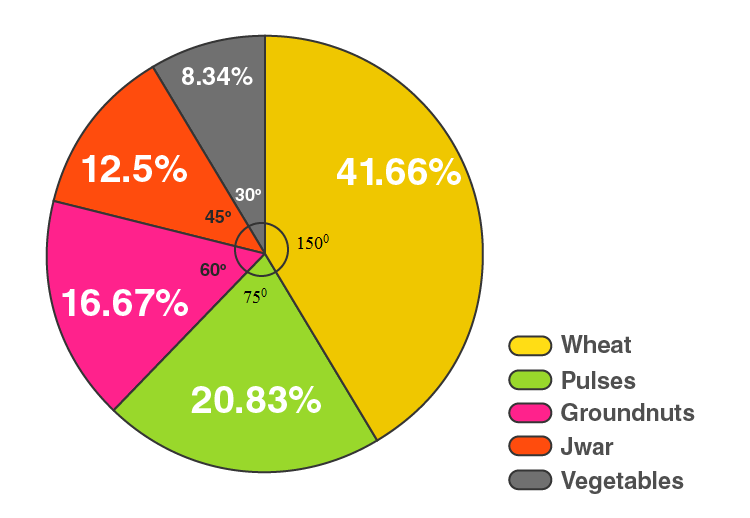8. Draw a pie diagram for the following data of expenditure pattern in a family:

 Items Food Clothing Rent Education Unforeseen events Medicine Expenditure (in %) 40% 20% 10% 10% 15% 5%

Solution:

Here, total % = 100%

So,

The central angle = (component value/100) × 360°

The central angle for each activity will be calculated as follows

 Items Expenditure (in %) Central angle Food 40% 40/100 × 360° = 144 Clothing 20% 20/100 × 360° = 72 Rent 10% 10/100 × 360° = 36 Education 10% 10/100 × 360° = 36 Unforeseen events 15% 15/100 × 360° = 54 Medicines 5% 5/100 × 360° = 18

Now, the pie-chart can be constructed by using the given data.

Steps to construct:

Step 1: Draw the circle of an appropriate radius.

Step 2: Draw a vertical radius anywhere inside the circle.

Step 3: Choose the largest central angle. Construct a sector of central angle, whose one radius coincides with the radius drawn in step 2 and the other radius is in clockwise direction to the vertical radius.

Step 4: Construct other sectors representing other values in clockwise direction in descending order of magnitudes of their central angles.

Step 5: Shade the sectors so obtained by different colours and label them as shown in below figure.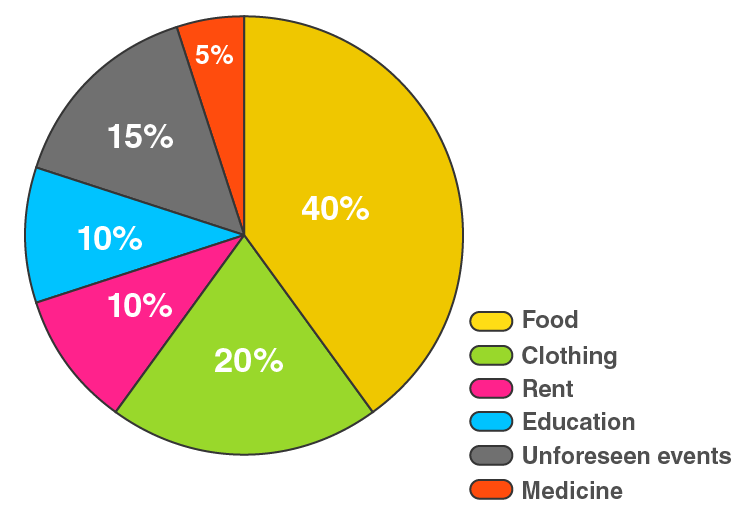9. Draw a pie diagram of the areas of continents of the world given in the following table:

 Continents Asia U.S.S.R Africa Europe North America South America Australia Area (in million sq.km) 26.9 20.5 30.3 4.9 24.3 17.9 8.5

Solution:

Here, total Area = 133.3 million sq.km

So,

The central angle = (component value/133.3) × 360°

The central angle for each activity will be calculated as follows

 Continents Area (in million sq.km) Central angle Asia 26.9 26.9/133.3 × 360° = 72.6 U.S.S.R 20.5 20.5/133.3 × 360° = 55.4 Africa 30.3 30.3/133.3 × 360° = 81.8 Europe 4.9 4.9/133.3 × 360° = 13.2 North America 24.3 24.3/133.3 × 360° = 65.6 South America 17.9 17.9/133.3 × 360° = 48.3 Australia 8.5 8.5/133.3 × 360° = 23

Now, the pie-chart can be constructed by using the given data.

Steps to construct:

Step 1: Draw the circle of an appropriate radius.

Step 2: Draw a vertical radius anywhere inside the circle.

Step 3: Choose the largest central angle. Construct a sector of central angle, whose one radius coincides with the radius drawn in step 2 and the other radius is in clockwise direction to the vertical radius.

Step 4: Construct other sectors representing other values in clockwise direction in descending order of magnitudes of their central angles.

Step 5: Shade the sectors so obtained by different colours and label them as shown in below figure.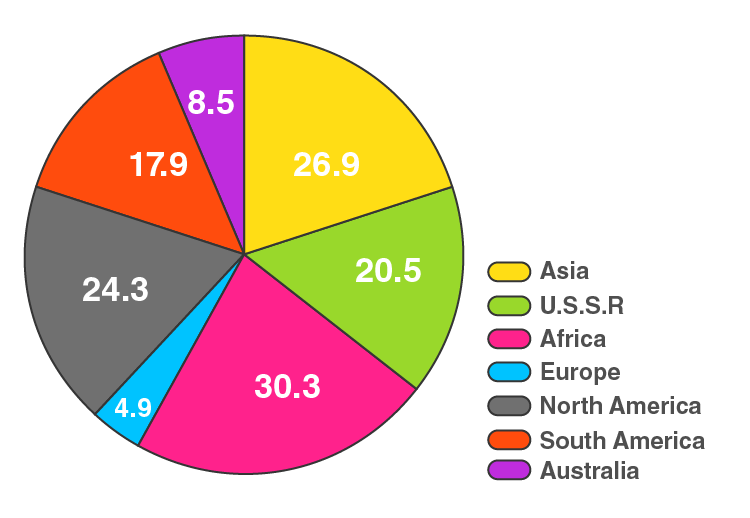10. The following data gives the amount spent on the construction of a house. Draw a pie diagram.

 Items Cement Timber Bricks Labour steel Miscellaneous Expenditure (in thousand Rs) 60 30 45 75 45 45

Solution:

Here, total Expenditure = 300 thousand rupees

So,

The central angle = (component value/300) × 360°

The central angle for each activity will be calculated as follows

 Items Expenditure (in thousand Rs) Central angle Cement 60 60/300 × 360° = 72 Timber 30 30/300 × 360° = 36 Bricks 45 45/300 × 360° = 54 Labour 75 75/300 × 360° = 90 Steel 45 45/300 × 360° = 54 Miscellaneous 45 45/300 × 360° = 54

Now, the pie-chart can be constructed by using the given data.

Steps to construct:

Step 1: Draw the circle of an appropriate radius.

Step 2: Draw a vertical radius anywhere inside the circle.

Step 3: Choose the largest central angle. Construct a sector of central angle, whose one radius coincides with the radius drawn in step 2 and the other radius is in clockwise direction to the vertical radius.

Step 4: Construct other sectors representing other values in clockwise direction in descending order of magnitudes of their central angles.

Step 5: Shade the sectors so obtained by different colours and label them as shown in below figure.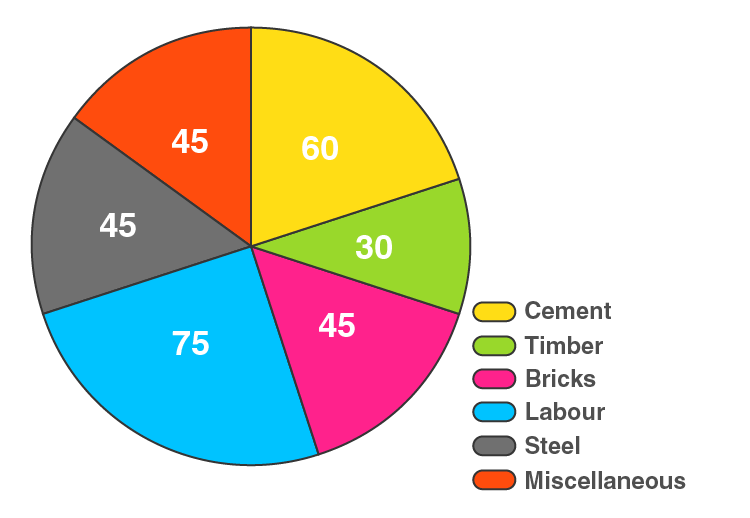11. The following table shows how a student spends his pocket money during the course of a month. Represent it by a pie diagram.

 Items Food Entertainment Other Expenditure Savings Expenditure 40% 25% 20% 15%

Solution:

Here, total Expenditure = 100%

So,

The central angle = (component value/100) × 360°

The central angle for each activity will be calculated as follows

 Items Expenditure Central angle Food 40% 40/100 × 360° = 144 Entertainment 25% 25/100 × 360° = 90 Other Expenditure 20% 20/100 × 360° = 72 Savings 15% 15/100 × 360° = 54

Now, the pie-chart can be constructed by using the given data.

Steps to construct:

Step 1: Draw the circle of an appropriate radius.

Step 2: Draw a vertical radius anywhere inside the circle.

Step 3: Choose the largest central angle. Construct a sector of central angle, whose one radius coincides with the radius drawn in step 2 and the other radius is in clockwise direction to the vertical radius.

Step 4: Construct other sectors representing other values in clockwise direction in descending order of magnitudes of their central angles.

Step 5: Shade the sectors so obtained by different colours and label them as shown in below figure.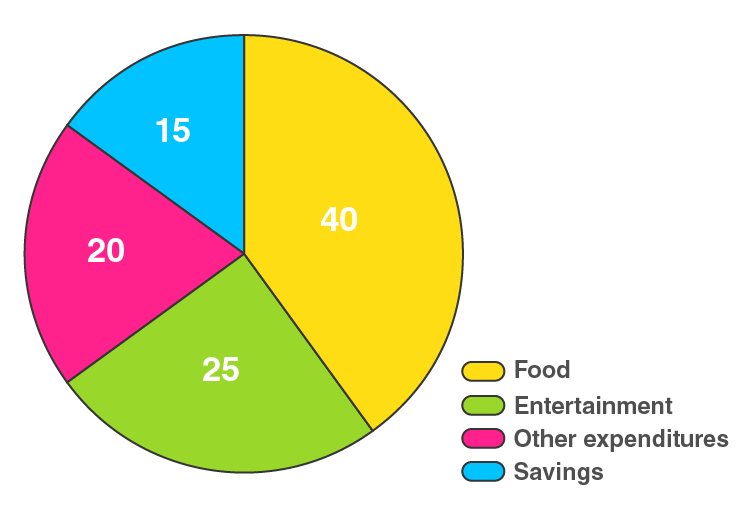12. Represent the following data by a pie diagram:

 Items of expenditure Expenditure Family A Family B Food 4000 6400 Clothing 2500 480 Rent 1500 3200 Education 400 1000 Miscellaneous 1600 600 Total 10000 16000

Solution:

Here, the total expenditure of family A = 10000 and family B = 11680

The central angle for family A = (component value/10000) × 360°

The central angle for family B = (component value/11680) × 360°

Hence, the central angle for each activity will be calculated as follows

 Items of expenditure Expenditure of Family A Expenditure of Family B Central angle of Family A Central angle of Family B Food 4000 6400 4000/10000 × 360° = 144 6400/11680 × 360° = 197.3 Clothing 2500 480 2500/10000 × 360° = 90 480/11680 × 360° = 14.8 Rent 1500 3200 1500/10000 × 360° = 54 3200/11680 × 360° = 98.6 Education 400 1000 400/10000 × 360° = 14.4 1000/11680 × 360° = 30.8 Miscellaneous 1600 600 1600/10000 × 360° = 57.6 600/11680 × 360° = 18.5

Now, the pie-chart for Family A and Family B can be constructed by using the given data.

Steps to construct:

Step 1: Draw the circle of an appropriate radius.

Step 2: Draw a vertical radius anywhere inside the circle.

Step 3: Choose the largest central angle. Construct a sector of central angle, whose one radius coincides with the radius drawn in step 2 and the other radius is in clockwise direction to the vertical radius.

Step 4: Construct other sectors representing other values in clockwise direction in descending order of magnitudes of their central angles.

Step 5: Shade the sectors so obtained by different colours and label them as shown in below figure.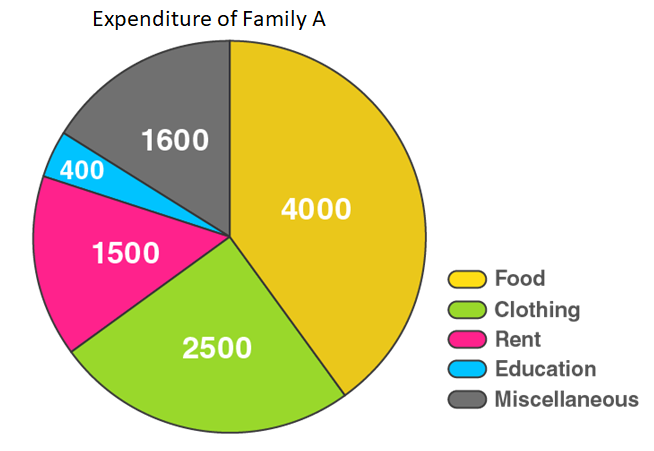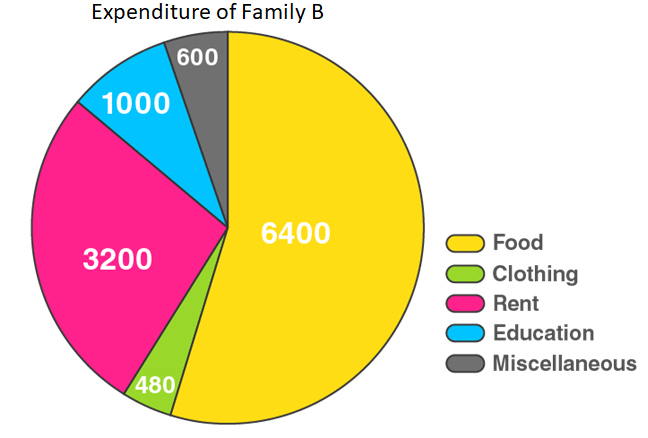13. Following data gives the break up of the cost of production of a book:

 Printing Paper Binding charges Advertisement Royalty Miscellaneous 30% 15% 15% 20% 10% 15%

Draw a pie-diagram depicting the above information.

Solution:

Here, total cost of production of book = 105%

So,

The central angle = (component value/105) × 360°

The central angle for each activity will be calculated as follows

 Items Expenditure Central angle Printing 30% 30/105 × 360° = 102.9 Paper 15% 15/105 × 360° = 51.4 Binding charges 15% 15/105 × 360° = 51.4 Advertisement 20% 20/105 × 360° = 68.6 Royalty 10% 10/105 × 360° = 34.3 Miscellaneous 15% 15/105 × 360° = 51.4

Now, the pie-chart can be constructed by using the given data.

Steps to construct:

Step 1: Draw the circle of an appropriate radius.

Step 2: Draw a vertical radius anywhere inside the circle.

Step 3: Choose the largest central angle. Construct a sector of central angle, whose one radius coincides with the radius drawn in step 2 and the other radius is in clockwise direction to the vertical radius.

Step 4: Construct other sectors representing other values in clockwise direction in descending order of magnitudes of their central angles.

Step 5: Shade the sectors so obtained by different colours and label them as shown in below figure.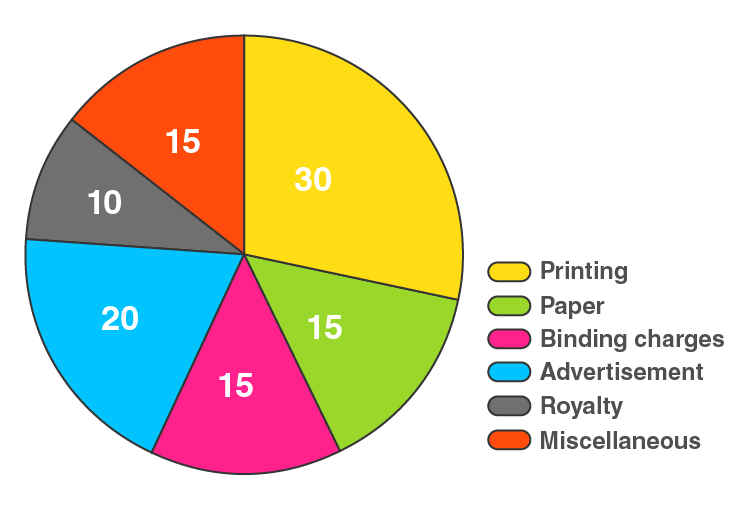14. Represent the following data with the help of pie diagram:

 Items Wheat Rice Tea Production (in metric tons) 3260 1840 900

Solution:

Here, total cost of production = 6000 metric tons

So,

The central angle = (component value/6000) × 360°

The central angle for each activity will be calculated as follows

 Items Production Central angle Wheat 3260 3260/6000 × 360° = 195.6 Rice 1840 1840/6000 × 360° = 110.4 Tea 900 900/6000 × 360° = 54

Now, the pie-chart can be constructed by using the given data.

Steps to construct:

Step 1: Draw the circle of an appropriate radius.

Step 2: Draw a vertical radius anywhere inside the circle.

Step 3: Choose the largest central angle. Construct a sector of central angle, whose one radius coincides with the radius drawn in step 2 and the other radius is in clockwise direction to the vertical radius.

Step 4: Construct other sectors representing other values in clockwise direction in descending order of magnitudes of their central angles.

Step 5: Shade the sectors so obtained by different colours and label them as shown in below figure.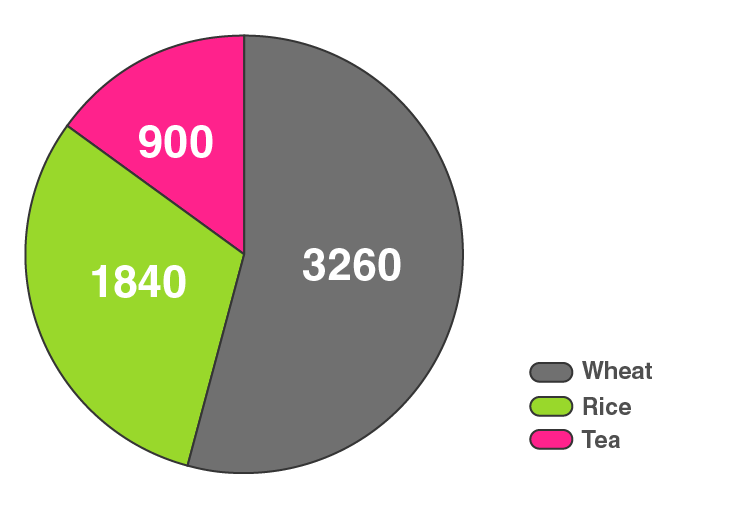15. Draw a pie-diagram representing the relative frequencies (expressed as percentage) of the eight classes as given below:
12.6, 18.2, 17.5, 20.3, 2.8, 4.2, 9.8, 14.7

Solution:

Here, total amount = 100.1%

So,

The central angle = (component value/100.1) × 360°

The central angle for each activity will be calculated as follows

 Class Amount (in %) Central angle 1 12.6 12.6/100.1 × 360° = 45.3 2 18.2 18.2/100.1 × 360° = 65.5 3 17.5 17.5/100.1 × 360° = 62.9 4 20.3 20.3/100.1 × 360° = 73 5 2.8 2.8/100.1 × 360° = 10.1 6 4.2 4.2/100.1 × 360° = 15.1 7 9.8 9.8/100.1 × 360° = 35.2 8 14.7 14.7/100.1 × 360° = 52.9

Now, the pie-chart can be constructed by using the given data.

Steps to construct:

Step 1: Draw the circle of an appropriate radius.

Step 2: Draw a vertical radius anywhere inside the circle.

Step 3: Choose the largest central angle. Construct a sector of central angle, whose one radius coincides with the radius drawn in step 2 and the other radius is in clockwise direction to the vertical radius.

Step 4: Construct other sectors representing other values in clockwise direction in descending order of magnitudes of their central angles.

Step 5: Shade the sectors so obtained by different colours and label them as shown in below figure.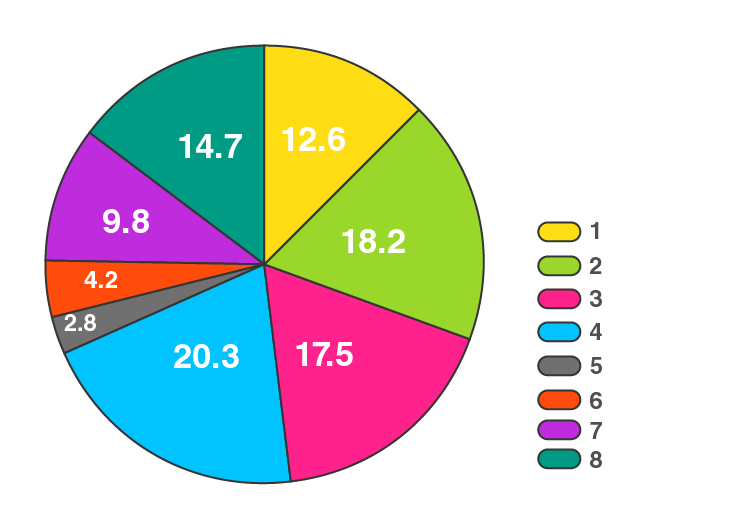16. Following is the break up of the expenditure of a family on different items of consumption:

 Items Food Clothing Rent Education Fuel etc. Medicine Miscellaneous Expenditure (in Rs) 1600 200 600 150 100 80 270

Draw a pie-diagram to represent the above data.

Solution:

Here, total expenditure = 3000 Rs

So,

The central angle = (component value/3000) × 360°

The central angle for each activity will be calculated as follows

 Items Expenditure (in Rs) Central angle Food 1600 1600/3000 × 360° = 192 Clothing 200 200/3000 × 360° = 24 Rent 600 600/3000 × 360° = 72 Education 150 150/3000 × 360° = 18 Fuel 100 100/3000 × 360° = 12 Medicine 80 80/3000 × 360° = 9.6 Miscellaneous 270 270/3000 × 360° = 32.4

Now, the pie-chart can be constructed by using the given data.

Steps to construct:

Step 1: Draw the circle of an appropriate radius.

Step 2: Draw a vertical radius anywhere inside the circle.

Step 3: Choose the largest central angle. Construct a sector of central angle, whose one radius coincides with the radius drawn in step 2 and the other radius is in clockwise direction to the vertical radius.

Step 4: Construct other sectors representing other values in clockwise direction in descending order of magnitudes of their central angles.

Step 5: Shade the sectors so obtained by different colours and label them as shown in below figure.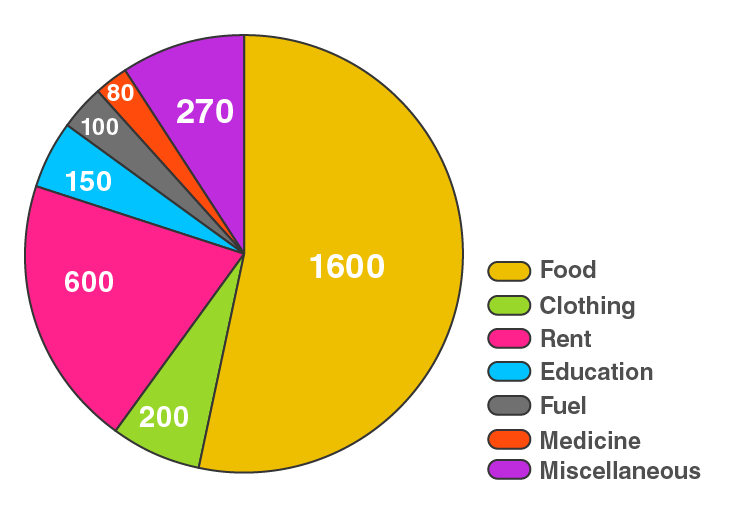17. Draw a pie diagram for the following data of the investment pattern in a five years plan:

 Agriculture Irrigation and Power Small Industries Transport Social service Miscellaneous 14% 16% 29% 17% 16% 8%

Solution:

Here, total investment = 100%

So,

The central angle = (component value/100) × 360°

The central angle for each activity will be calculated as follows

 Data Investment Central angle Agriculture 14% 14/100 × 360° = 50.4 Irrigation and Power 16% 16/100 × 360° = 57.6 Small Industries 29% 29/100 × 360° = 104.4 Transport 17% 17/100 × 360° = 61.2 Social service 16% 16/100 × 360° = 57.6 Miscellaneous 8% 8/100 × 360° = 28.8

Now, the pie-chart can be constructed by using the given data.

Steps to construct:

Step 1: Draw the circle of an appropriate radius.

Step 2: Draw a vertical radius anywhere inside the circle.

Step 3: Choose the largest central angle. Construct a sector of central angle, whose one radius coincides with the radius drawn in step 2 and the other radius is in clockwise direction to the vertical radius.

Step 4: Construct other sectors representing other values in clockwise direction in descending order of magnitudes of their central angles.

Step 5: Shade the sectors so obtained by different colours and label them as shown in below figure.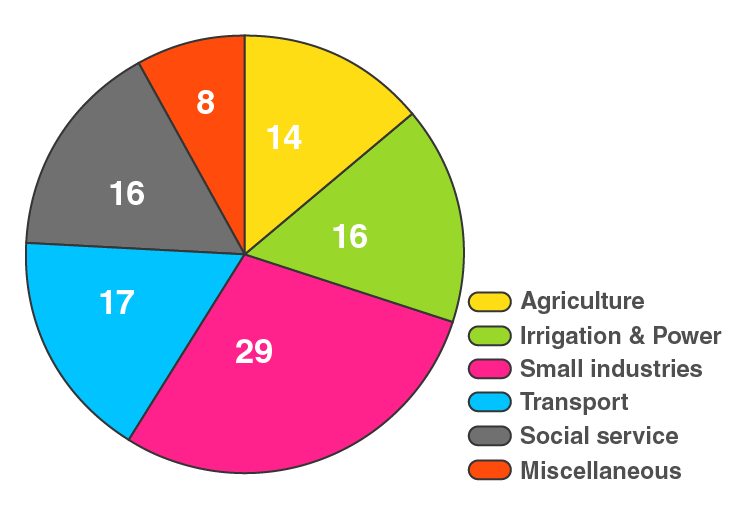EXERCISE 25.2 PAGE NO: 25.21

1. The pie chart given in Fig. 25.17 represents the expenditure on different items in constructing a flat in Delhi. If the expenditure incurred on cement is Rs. 112500, find the following: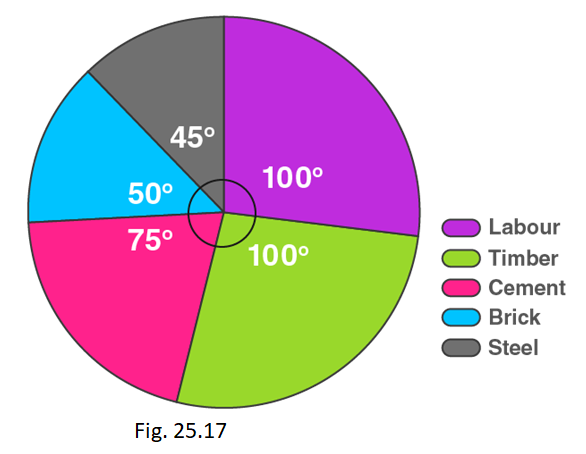(i) Total cost of the flat.
(ii) Expenditure incurred on labour.

Solution:

(i) By using the formula,

Expenditure incurred on cement = (central angle × Total cost) / 360°

Total cost of the flat = (360° × 112500) / 75o = Rs 540000

(ii) By using the formula,

Expenditure incurred on labour = (central angle × Total cost) / 360°

= (100° × 540000) / 360o = Rs 150000

2. The pie-chart given in Fig. 25.18 shows the annual agricultural production of an Indian state. If the total production of all the commodities is 81000 tonnes, find the production (in tonnes) of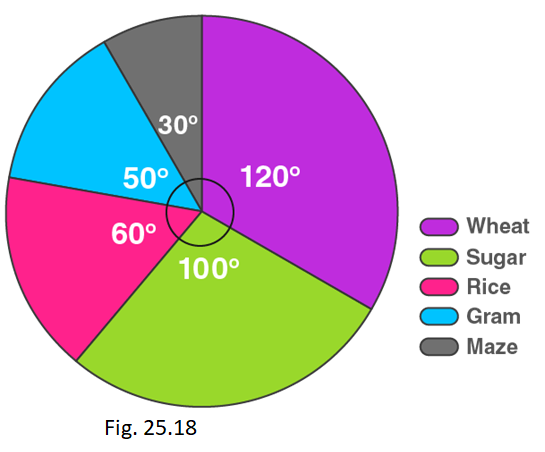(i) Wheat (ii) Sugar (iii) Rice (iv) Maize (v) Gram

Solution:

We know that,

Total Production = 81000 Tonnes.

So,

(i) Production of wheat = (central angle of wheat × Total production) / 360°

= (120o × 81000) / 360o = 27000 tonnes

(ii) Production of sugar = (central angle of sugar × Total production) / 360°

= (100o × 81000) / 360o = 22500 tonnes

(iii) Production of rice = (central angle of rice × Total production) / 360°

= (60o × 81000) / 360o = 13500 tonnes

(iv) Production of maize = (central angle of maize × Total production) / 360°

= (30o × 81000) / 360o = 6750 tonnes

(v) Production of gram = (central angle of gram × Total production) / 360°

= (50o × 81000) / 360o = 11250 tonnes

3. The following pie chart shows the number of students admitted in different faculties of a college. If 1000 students are admitted in Science answer the following :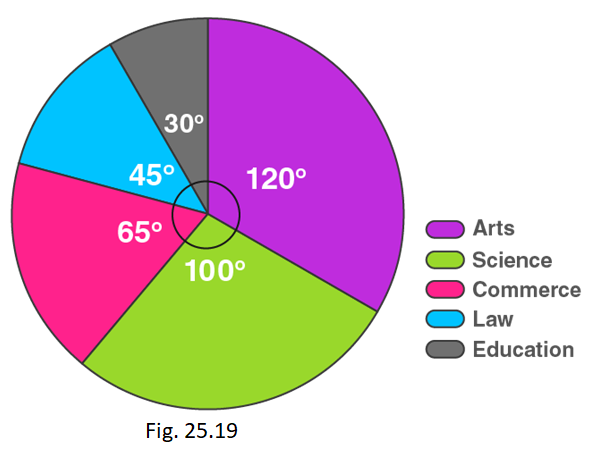(i) What is the total number of students?
(ii) What is the ratio of students in science and arts?

Solution:

(i)

Students in science = (central angle × Total students) / 360°

1000 = (100o × Total students) / 360o

Total students = (1000 × 360o)/100o

= 3600 students

∴ Total number of students are 3600.

(ii) Students in arts = (central angle of arts × Total students) / 360°

= (120o × 3600) / 360o = 1200 students

∴ Ratio of students in science and arts is 1000:1200 = 5:6

4. In Fig. 25.20, the pie-chart shows the marks obtained by a student in an examination. If the student secures 440 marks in all, calculate his marks in each of the given subjects.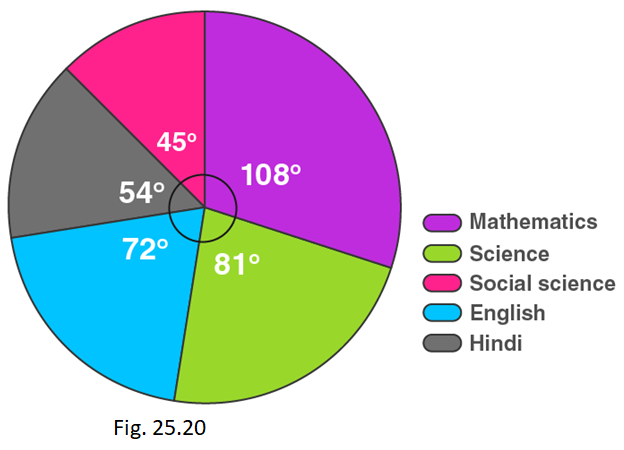Solution:

Marks secured in mathematics = (central angle of maths × Total score secured) / 360°

= (108 × 440) / 360o = 132 marks

Marks secured in science = (central angle of science × Total score secured) / 360°

= (81 × 440) / 360o = 99 marks

Marks secured in English = (central angle of English × Total score secured) / 360°

= (72 × 440) / 360o = 88 marks

Marks secured in Hindi = (central angle of Hindi × Total score secured) / 360°

= (54 × 440) / 360o = 66 marks

Marks secured in social science = (central angle of social science × Total score secured) / 360°

= (45 × 440) / 360o = 55 marks

 Subject Mathematics Science English Hindi Social Science Marks secured 132 99 88 66 55

5. In Fig. 25.21, the pie chart shows the marks obtained by a student in various subjects. If the student scored 135 marks in mathematics, find the total marks in all the subjects. Also, find his score in individual subjects.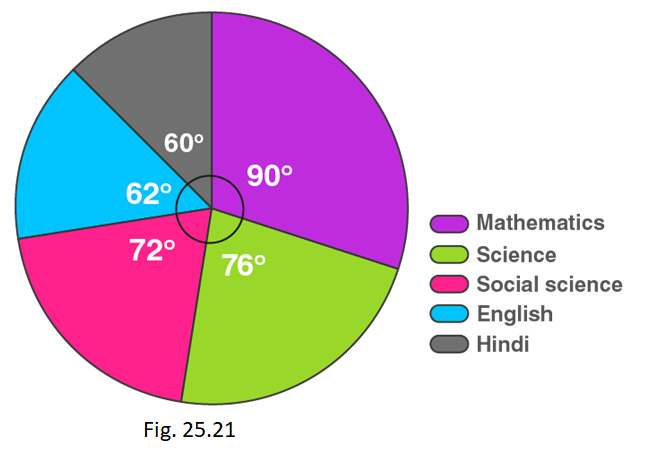Solution:

Let us calculate the total marks.

So,

Marks scored in mathematics = (central angle of maths × Total marks) / 360°

135 = (90 × Total marks) / 360o

Total marks = (135 × 360)/90

= 540 marks

Now,

Marks scored in Hindi = (central angle of Hindi × Total marks) / 360°

= (60 × 540) / 360o

= 90 marks

Marks scored in Science = (central angle of Science × Total marks) / 360°

= (76 × 540) / 360o

= 114 marks

Marks scored in Social science = (central angle of Social science × Total marks) / 360°

= (72 × 540) / 360o

= 108 marks

Marks scored in English = (central angle of English × Total marks) / 360°

= (62 × 540) / 360o

= 93 marks

 Subject Mathematics Science Social science English Hindi Marks secured 135 114 108 93 90

6. The following pie chart shows the monthly expenditure of Shikha on various items. If she spends Rs 16000 per month, answer the following questions: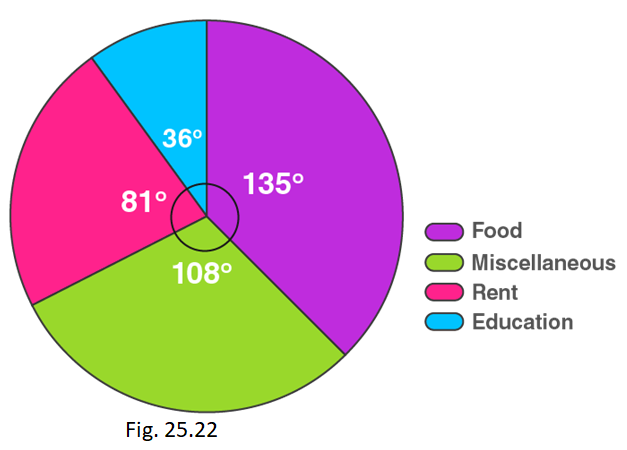(i) How much does she spend on rent?

(ii) How much does she spend on education?

(iii) What is the ratio of expenses on food and rent?

Solution:

(i) Money spent on rent = (central angle of rent × Total money spent) / 360°

= (81 × 16000) / 360o

= Rs 3600

(ii) Money spent on education = (central angle of education × Total money spent) / 360°

= (36 × 16000) / 360o

= Rs 1600

(iii) Money spent on food = (central angle of food × Total money spent) / 360°

= (135 × 16000) / 360o

= Rs 6000

Ratio of expenses on food and rent is Rs 6000/Rs3600 = 5/3

Ratio = 5:3

7. The pie chart (as shown in the figure 25.23) represents the amount spent on different sports by a sports club in a year. If the total money spent by the club on sports is Rs 108000, find the amount spent on each sport.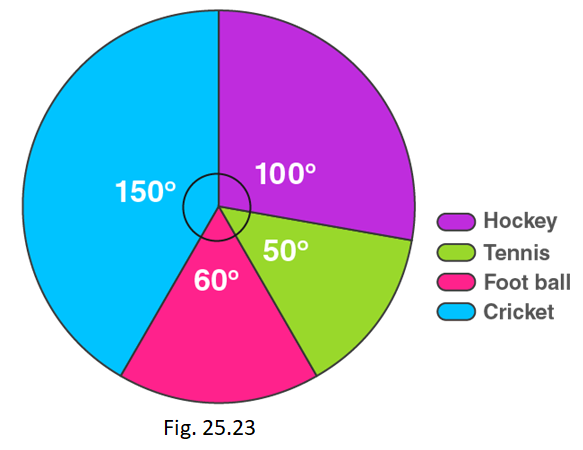Solution:

Money spent on cricket = (central angle of cricket × Total money spent) / 360°

= (150 × 108000) / 360o

= Rs 45000

Money spent on football = (central angle of football × Total money spent) / 360°

= (60 × 108000) / 360o

= Rs 18000

Money spent on tennis = (central angle of tennis × Total money spent) / 360°

= (50 × 108000) / 360o

= Rs 15000

Money spent on hockey = (central angle of cricket × Total money spent) / 360°

= (100 × 108000) / 360o

= Rs 30000